# JP5853097B2 - Three-phase synchronous motor drive device, integrated three-phase synchronous motor, positioning device and pump device - Google Patents

Three-phase synchronous motor drive device, integrated three-phase synchronous motor, positioning device and pump device Download PDF

## Info

Publication number
JP5853097B2
JP5853097B2 JP2014509989A JP2014509989A JP5853097B2 JP 5853097 B2 JP5853097 B2 JP 5853097B2 JP 2014509989 A JP2014509989 A JP 2014509989A JP 2014509989 A JP2014509989 A JP 2014509989A JP 5853097 B2 JP5853097 B2 JP 5853097B2
Authority
JP
Japan
Prior art keywords
phase
synchronous motor
rotor
phase synchronous
switch
Prior art date
Legal status (The legal status is an assumption and is not a legal conclusion. Google has not performed a legal analysis and makes no representation as to the accuracy of the status listed.)
Active
Application number
JP2014509989A
Other languages
Japanese (ja)
Other versions
JPWO2013153657A1 (en
Inventor

Original Assignee

Priority date (The priority date is an assumption and is not a legal conclusion. Google has not performed a legal analysis and makes no representation as to the accuracy of the date listed.)
Filing date
Publication date
Application filed by 株式会社日立製作所 filed Critical 株式会社日立製作所
Priority to PCT/JP2012/060040 priority Critical patent/WO2013153657A1/en
Publication of JPWO2013153657A1 publication Critical patent/JPWO2013153657A1/en
Application granted granted Critical
Publication of JP5853097B2 publication Critical patent/JP5853097B2/en
Active legal-status Critical Current
Anticipated expiration legal-status Critical

• 230000001360 synchronised Effects 0.000 title claims description 116
• 230000001264 neutralization Effects 0.000 claims description 187
• 230000003334 potential Effects 0.000 claims description 76
• 244000171263 Ribes grossularia Species 0.000 claims description 62
• 230000004907 flux Effects 0.000 claims description 30
• 238000004804 winding Methods 0.000 claims description 7
• 239000007788 liquids Substances 0.000 claims description 2
• 239000000969 carriers Substances 0.000 description 55
• 238000010586 diagrams Methods 0.000 description 52
• 241001442055 Vipera berus Species 0.000 description 27
• 230000015654 memory Effects 0.000 description 24
• 238000004364 calculation methods Methods 0.000 description 19
• 230000000875 corresponding Effects 0.000 description 14
• 238000006243 chemical reactions Methods 0.000 description 10
• 238000000034 methods Methods 0.000 description 7
• 230000000630 rising Effects 0.000 description 7
• 239000003921 oils Substances 0.000 description 6
• 230000000051 modifying Effects 0.000 description 5
• 239000003570 air Substances 0.000 description 3
• 238000004378 air conditioning Methods 0.000 description 3
• 230000001276 controlling effects Effects 0.000 description 3
• 239000000203 mixtures Substances 0.000 description 3
• 238000005070 sampling Methods 0.000 description 3
• 230000035945 sensitivity Effects 0.000 description 3
• 280000358881 Magnet, Inc. companies 0.000 description 2
• 230000000694 effects Effects 0.000 description 2
• 230000004044 response Effects 0.000 description 2
• 239000000758 substrates Substances 0.000 description 2
• 238000005406 washing Methods 0.000 description 2
• 230000003321 amplification Effects 0.000 description 1
• 230000005540 biological transmission Effects 0.000 description 1
• 238000004891 communication Methods 0.000 description 1
• 238000001514 detection method Methods 0.000 description 1
• 230000005284 excitation Effects 0.000 description 1
• 230000001771 impaired Effects 0.000 description 1
• 238000003199 nucleic acid amplification method Methods 0.000 description 1
• 230000004043 responsiveness Effects 0.000 description 1
• 230000001629 suppression Effects 0.000 description 1
• 230000001131 transforming Effects 0.000 description 1
• 239000011901 water Substances 0.000 description 1
• 230000003313 weakening Effects 0.000 description 1

## Images

•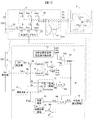•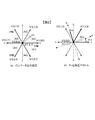•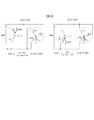•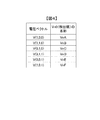•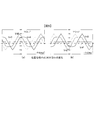•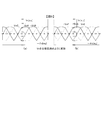•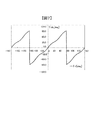••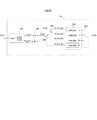•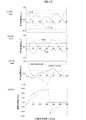•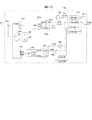•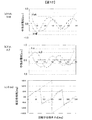•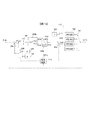•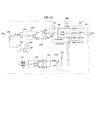•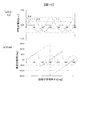•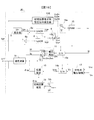••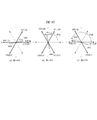•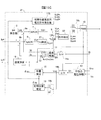••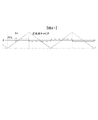•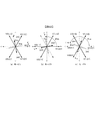•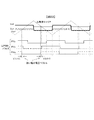•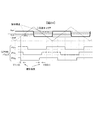•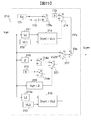••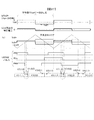•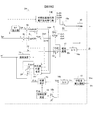•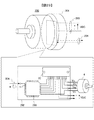•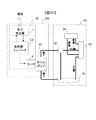•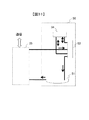•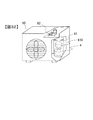•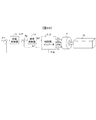•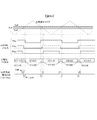•## Classifications

• HELECTRICITY
• H02GENERATION; CONVERSION OR DISTRIBUTION OF ELECTRIC POWER
• H02PCONTROL OR REGULATION OF ELECTRIC MOTORS, ELECTRIC GENERATORS OR DYNAMO-ELECTRIC CONVERTERS; CONTROLLING TRANSFORMERS, REACTORS OR CHOKE COILS
• H02P6/00Arrangements for controlling synchronous motors or other dynamo-electric motors using electronic commutation dependent on the rotor position; Electronic commutators therefor
• H02P6/14Electronic commutators
• H02P6/16Circuit arrangements for detecting position
• H02P6/18Circuit arrangements for detecting position without separate position detecting elements
• H02P6/182Circuit arrangements for detecting position without separate position detecting elements using back-emf in windings
• HELECTRICITY
• H02GENERATION; CONVERSION OR DISTRIBUTION OF ELECTRIC POWER
• H02PCONTROL OR REGULATION OF ELECTRIC MOTORS, ELECTRIC GENERATORS OR DYNAMO-ELECTRIC CONVERTERS; CONTROLLING TRANSFORMERS, REACTORS OR CHOKE COILS
• H02P6/00Arrangements for controlling synchronous motors or other dynamo-electric motors using electronic commutation dependent on the rotor position; Electronic commutators therefor
• H02P6/14Electronic commutators
• H02P6/16Circuit arrangements for detecting position
• H02P6/18Circuit arrangements for detecting position without separate position detecting elements
• HELECTRICITY
• H02GENERATION; CONVERSION OR DISTRIBUTION OF ELECTRIC POWER
• H02PCONTROL OR REGULATION OF ELECTRIC MOTORS, ELECTRIC GENERATORS OR DYNAMO-ELECTRIC CONVERTERS; CONTROLLING TRANSFORMERS, REACTORS OR CHOKE COILS
• H02P6/00Arrangements for controlling synchronous motors or other dynamo-electric motors using electronic commutation dependent on the rotor position; Electronic commutators therefor
• H02P6/14Electronic commutators
• H02P6/16Circuit arrangements for detecting position
• H02P6/18Circuit arrangements for detecting position without separate position detecting elements
• H02P6/183Circuit arrangements for detecting position without separate position detecting elements using an injected high frequency signal
• HELECTRICITY
• H02GENERATION; CONVERSION OR DISTRIBUTION OF ELECTRIC POWER
• H02PCONTROL OR REGULATION OF ELECTRIC MOTORS, ELECTRIC GENERATORS OR DYNAMO-ELECTRIC CONVERTERS; CONTROLLING TRANSFORMERS, REACTORS OR CHOKE COILS
• H02P6/00Arrangements for controlling synchronous motors or other dynamo-electric motors using electronic commutation dependent on the rotor position; Electronic commutators therefor
• H02P6/14Electronic commutators
• H02P6/16Circuit arrangements for detecting position
• H02P6/18Circuit arrangements for detecting position without separate position detecting elements
• H02P6/187Circuit arrangements for detecting position without separate position detecting elements using the star point voltage

## Description

The present invention relates to a three-phase synchronous motor driving device , an integrated three-phase synchronous motor including the three-phase synchronous motor driving device, a positioning device, a pump device, and the like.

Small and highly efficient permanent magnet motors (three-phase synchronous motors) are widely used in various fields such as industrial equipment, home appliances, and automobiles. However, in order to drive the permanent magnet motor, position information of the rotor of the motor is required, and a position sensor for that purpose is required.

In recent years, sensorless control that eliminates this position sensor and performs rotation speed and torque control of a permanent magnet motor has become widespread. By putting sensorless control into practical use, it is possible to reduce the cost of the position sensor (the cost of the sensor itself, the cost of sensor wiring, etc.) and the size of the device. Further, since the sensor is not necessary, there is an advantage that it can be used in a poor environment. Currently, sensorless control of a permanent magnet motor is a method of directly detecting an induced voltage (speed electromotive voltage) generated by rotation of a rotor of a permanent magnet motor and driving the permanent magnet motor as position information of the rotor. A position estimation technique for estimating and calculating the rotor position from a mathematical model of the target motor is employed.

These sensorless controls also have major challenges. It is a position detection method during low speed operation. Most sensorless control currently in practical use is based on an induced voltage generated by a permanent magnet motor. Therefore, at the time of stopping or in a low speed region where the induced voltage is small, the sensitivity is lowered, and the position information may be buried in noise. Various solutions have been proposed for this problem.

The invention described in Patent Document 1 obtains position information by detecting a “neutral point potential” that is a potential at a connection point of a three-phase stator winding. By detecting this neutral point potential in synchronization with the pulse voltage applied from the inverter to the motor, an electromotive voltage due to inductance imbalance can be detected, and a potential change depending on the rotor position can be obtained. Therefore, the position information can be obtained by PWM (pulse width modulation) at the time of normal sine wave modulation as the voltage applied to the motor. Here, the rotor position means the position of the permanent magnet incorporated in the rotor.

JP 2010-74889 A

However, when trying to estimate the rotor position of the motor by the method described in Patent Document 1 described above, only the position estimation of a half cycle (± 90 deg) can be performed with respect to one electrical angle cycle. Thus, the magnetic polarity cannot be determined. Therefore, if the motor is started immediately after the inverter power is turned on, the estimated rotor position may include an error of 180 deg, and may rotate in the reverse direction with a probability of 1/2. was there.

According to the first aspect of the present invention, a three-phase synchronous motor driving device includes a switching element for three phases, a three-phase inverter that drives the three-phase synchronous motor, and an on / off state of the switching element for three phases. Four switch states are selected from a plurality of switch states to be expressed, and the neutral point potential of the control unit for sequentially controlling the three-phase inverter in the four switch states and the stator winding of the three-phase synchronous motor is set to 4 The rotor position of the three-phase synchronous motor is electrically detected based on at least three of the neutral point potential detection unit that detects each of the four switch states and the four neutral point potentials that are detected in the four switch states. A first rotor position estimator that estimates within a range of one angular period, and the four switch vectors representing the four switch states are the first switch vector and the second And switch vector, and a reverse of the third switch vector and the fourth switch vector each other.
According to the second aspect of the present invention, in the three-phase synchronous motor drive device according to the first aspect, the control unit outputs a first three-phase voltage command for initial position estimation that indicates four switch states, A voltage command output unit that outputs at the time of rotation start of the phase synchronous motor is provided, and the first rotor position estimation unit is detected when the first three-phase voltage command is output from the voltage command output unit. It is preferable to estimate the rotor position at the start of rotation based on the neutral point potential.
According to the third aspect of the present invention, in the three-phase synchronous motor drive device according to the second aspect, the voltage command output unit further includes the first rotor position estimation unit after the output of the first three-phase voltage command. The second three-phase voltage command is output based on the rotor position estimated by the following equation. The second three-phase voltage command includes four switch vectors, two of which sandwich the positive direction of the rotor magnetic flux vector. It is preferable that the three-phase voltage command indicates four switch states such that the vector and two vectors sandwiching the negative direction of the rotor magnetic flux vector.
According to the fourth aspect of the present invention, in the three-phase synchronous motor drive apparatus according to the second or third aspect, there are four third three-phase voltage commands generated based on the phase current information of the three-phase synchronous motor. Rotational torque voltage command generated by the control unit so as to be a voltage command for instructing a switch state of the motor and a voltage command for instructing a vector having a relationship adjacent to the rotor magnetic flux vector as four switch vectors. It is preferable that a first voltage command correction unit for correcting the voltage is further provided, and the control unit controls the three-phase inverter based on the rotational torque voltage command corrected by the first voltage command correction unit.
According to the fifth aspect of the present invention, in the three-phase synchronous motor drive device according to the fourth aspect, the third three-phase voltage command generated based on the phase current information of the three-phase synchronous motor has four switches. For the rotational torque generated by the controller so that it becomes a voltage command that indicates a state and a voltage command that indicates a vector adjacent to a vector orthogonal to the rotor magnetic flux vector as four switch vectors A second voltage command correction unit configured to correct the voltage command, and the control unit, when the magnitude of the rotation torque voltage command is smaller than a predetermined value, the rotation torque corrected by the second voltage command correction unit; Based on the voltage command, the three-phase inverter is controlled, and if the magnitude of the voltage command for rotation torque is equal to or greater than a predetermined value, the voltage command for rotation torque corrected by the first voltage command correction unit Based on, it is preferable to control the three-phase inverter.
According to the sixth aspect of the present invention, in the three-phase synchronous motor drive device according to the fourth or fifth aspect, the difference between the voltage commands of each phase in the third three-phase voltage command is larger than the predetermined difference value. A third voltage command correction unit that corrects so as to be satisfied is provided.
According to the seventh aspect of the present invention, in the three-phase synchronous motor drive device according to any one of the fourth to sixth aspects, two neutral point potentials among the four neutral point potentials or the stator Based on the induced voltage induced in the windings, the second rotor position estimation unit that estimates the rotor position of the three-phase synchronous motor, and the rotor estimated by the first or second rotor position estimation unit A rotation speed determination unit that determines whether the rotation speed of the three-phase synchronous motor is higher than a predetermined rotation speed based on the position, and the control unit determines that the rotation speed is higher than the predetermined rotation speed. The three-phase inverter is controlled by the four switch states, and when the rotational speed determination unit determines that the rotational speed is equal to or lower than the predetermined rotational speed, the three-phase inverter is preferably controlled by two of the four switch states. .
According to the eighth aspect of the present invention, in the three-phase synchronous motor drive device according to any one of the fourth to sixth aspects, the control unit has four ways when the voltage output from the three-phase inverter is equal to or less than a predetermined value. It is preferable to control the three-phase inverter by two of the four switch states when the three-phase inverter is controlled by the switch state and the voltage output from the three-phase inverter is greater than a predetermined value.
According to the ninth aspect of the present invention, in the three-phase synchronous motor drive apparatus according to any one of the second to eighth aspects, the first rotor position estimating unit is detected in the first and second switch vectors. The sum of neutral point potentials and the sum of neutral point potentials detected in the third and fourth switch vectors are calculated, and the rotor position of the three-phase synchronous motor is estimated based on the two calculated sums. It is preferable to do this.
According to a tenth aspect of the present invention, in the three-phase synchronous motor drive device according to any one of the second to eighth aspects, the first rotor position estimating unit is directed in the same direction among the four switch vectors. A first position information acquisition unit that obtains a first rotor position information based on the difference between the neutral point potentials of the two switch vectors, and the first and second switch vectors. And the neutral point potential detected in the third and fourth switch vectors is calculated, and the second rotor position information is calculated based on the two calculated sums. A polarity discriminating unit, and a polarity discriminating unit that discriminates the magnetic flux polarity of the rotor position of the three-phase synchronous motor based on the first and second rotor position information. Discrimination result and first rotor position information Based on the bets, preferably to estimate the rotor position of the three-phase synchronous motor.
According to an eleventh aspect of the present invention, in the three-phase synchronous motor driving device according to any one of the second to eighth aspects, the first rotor position estimating unit is directed in the same direction among the four switch vectors. The first position information acquisition unit that obtains the difference between the neutral point potentials in the two switch vectors and obtains the first rotor position information based on the difference, one of the two switch vectors, and one of them Magnetic flux at the rotor position of the three-phase synchronous motor is acquired based on the sum of the two neutral point potentials and the first rotor position information. And a polarity discriminating unit for discriminating the polarity, and estimating the rotor position of the three-phase synchronous motor based on the discrimination result of the polarity discriminating unit and the first rotor position information.
According to a twelfth aspect of the present invention, in the three-phase synchronous motor drive apparatus according to any one of the second to eighth aspects, the first rotor position estimating unit is detected in the first and second switch vectors. The sum of neutral point potentials and the sum of neutral point potentials detected in the third and fourth switch vectors are calculated, and second rotor position information is acquired based on the two calculated sums. Calculating a second position information acquisition unit, a difference between neutral point potentials detected in the first and second switch vectors, and a difference between neutral point potentials detected in the third and fourth switch vectors; A third position information acquisition unit that acquires third rotor position information based on the difference between the two, and a magnetic flux at the rotor position of the three-phase synchronous motor based on the second and third rotor position information A polarity discriminator for discriminating the polarity The equipped, on the basis of the determination result and the third rotor position information polarity determination unit preferably estimates the rotor position of the three-phase synchronous motor in the electrical angle one cycle range.
According to a thirteenth aspect of the present invention, an integrated three-phase synchronous motor includes a three-phase synchronous motor drive device according to any one of the second to twelfth aspects, and three homologues controlled by the three-phase synchronous motor drive device. The rotor and stator of the motor are housed in a common housing.
According to a fourteenth aspect of the present invention, a positioning device includes a three-phase synchronous motor drive device according to any one of the second to twelfth aspects, a three-phase synchronous motor driven and controlled by the three-phase synchronous motor drive device, And a positioning stage that is driven to slide or rotate when the three-phase synchronous motor rotates forward and backward.
According to a fifteenth aspect of the present invention, a pump device includes the three-phase synchronous motor drive device according to any one of the second to twelfth aspects, a three-phase synchronous motor driven and controlled by the three-phase synchronous motor drive device, A liquid pump driven by a three-phase synchronous motor.

According to the present invention, the rotor position of a three-phase synchronous motor in a stopped state can be estimated within a range of one electrical angle cycle, and sensorless driving with a sinusoidal current can be realized immediately from the stopped state.

FIG. 1 is a diagram for explaining a first embodiment of a three-phase synchronous motor driving apparatus according to the present invention. FIG. 2 is a diagram for explaining a voltage vector (switch vector). FIG. 3 is a diagram illustrating the neutral point potential. FIG. 4 is a diagram showing the relationship between the voltage vector and the neutral point potential. FIG. 5 is a diagram showing changes in neutral point potentials VnA, VnB, VnC, VnD, VnE, and VnF with respect to the rotor position (phase) θd. FIG. 6 is a diagram showing changes in neutral point potentials VnA, -VnB, VnC, -VnD, VnE, and -VnF. FIG. 7 is a diagram illustrating θdc when the rotor position is estimated using neutral point potentials detected with respect to two voltage vectors. FIG. 8 is a diagram showing the three-phase voltage commands Vu0 *, Vv0 *, Vw0 *, PWM pulse, voltage vector, and neutral point potential Vn0 in the first embodiment. FIG. 9 is a block diagram of the initial position estimator 19. FIG. 10 is a diagram illustrating waveforms and estimated phase angles θds of VnA, VnB, VnD, VnE, VnU, and VnW. FIG. 11 is a block diagram of the initial position estimator 19B in the second embodiment. FIG. 12 is a diagram illustrating waveforms of VnA, VnB, Xα, and Xβ and an estimated phase angle θds0 in the initial position estimator 19B. FIG. 13 is a block diagram of an initial position estimator 19C in the third embodiment. FIG. 14 is a block diagram of an initial position estimator 19D in the fourth embodiment. FIG. 15 is a diagram illustrating Xα, Xβ, θds0 in the fourth embodiment. FIG. 16 is a block diagram of the controller 2E of the fifth embodiment. FIG. 17 is a diagram showing a configuration of the initial position estimation voltage command generator 17E. FIG. 18 is a vector diagram showing the relationship between the four voltage vectors and the rotor position. FIG. 19 is a block diagram of the controller 2F of the sixth embodiment. FIG. 20 is a diagram illustrating a configuration of the Vq corrector 21. FIG. 21 is a diagram illustrating a waveform of the signal dVq. FIG. 22 is a diagram showing an applied voltage vector when Vq ** is used. FIG. 23 is a diagram showing a PWM pulse waveform before correction by the three-phase corrector 22. FIG. 24 is a diagram illustrating a PWM pulse waveform after correction by the three-phase corrector 22. FIG. 25 is a block diagram of the Vq corrector 21G of the seventh embodiment. FIG. 26 is a diagram for explaining selection of a voltage vector when the voltage command Vq * is “positive”. FIG. 27 is a diagram for explaining selection of a voltage vector when the voltage command Vq * is “negative”. FIG. 28 is a diagram illustrating a configuration of the controller 2H according to the eighth embodiment. FIG. 29 is a diagram illustrating an integrated three-phase synchronous motor according to the ninth embodiment. FIG. 30 is a diagram illustrating a pump device 300 according to the tenth embodiment. FIG. 31 is a diagram showing a configuration in which the relief valve is removed from the pump device 300 shown in FIG. FIG. 32 is a diagram illustrating a compressor drive system according to the eleventh embodiment. FIG. 33 is a diagram illustrating an overall block configuration of the positioning device according to the twelfth embodiment. FIG. 34 is a diagram showing a PWM waveform, a neutral point potential waveform, and the like in the conventional PWM control. FIG. 35 is a block diagram of the Vq corrector 21H according to the eighth embodiment.

Hereinafter, embodiments for carrying out the present invention will be described with reference to the drawings. The three-phase synchronous motor driving device according to the present invention includes a rotational speed control for a fan, a pump (hydraulic pump, water pump), a compressor, a washing machine, a spindle motor, a disk driver, etc., and a positioning device for a conveyor or a machine tool. In addition, it can be used for applications that control torque, such as electric assist.

-First embodiment-
FIG. 1 is a diagram for explaining a first embodiment of a three-phase synchronous motor driving apparatus according to the present invention. The drive control device 100 is a device that drives a permanent magnet motor (hereinafter referred to as a motor) 4 that is a three-phase synchronous motor, and includes an Iq * generator 1, a controller 2, an inverter main circuit 32, and a one-shunt current. An inverter 3 including a detector 35 is provided. The inverter 3 is connected to a DC power source 31.

The Iq * generator 1 is a circuit that generates a current command Iq * corresponding to the torque of the motor 4. The Iq * generator 1 is a controller positioned above the controller 2. Here, the Iq * generator 1 is also included in the drive control device 100, but may be configured not to be included. Usually, the necessary current command Iq * is generated while observing the actual speed ω1 so that the rotation speed of the motor 4 becomes a predetermined speed. The current command Iq *, which is the output of the Iq * generator 1, is output to the subtractor 6 b provided in the controller 2.

The controller 2 operates so that the motor 4 generates a torque corresponding to the current command Iq *. The controller 2 includes an Id * generator (d-axis current command generator) 5, a subtractor 6a, a subtractor 6b, a d-axis current controller (IdACR) 7, a q-axis current controller (IqACR) 8, and a dq inverse. Converter 9, PWM generator 10, current reproducer 11, dq converter 12, neutral point potential amplifier 13, sample / hold circuits 14a and 14b, position estimator 15, speed calculator 16, initial position estimation voltage command A generator 17, initial position estimation changeover switches 18a and 18b, and an initial position estimator 19 are provided.

The inverter 3 includes an output pre-driver 33 and a virtual neutral point circuit 34 in addition to the inverter main circuit 32 and the one-shunt current detector 35 described above. The DC power supply 31 is a DC power supply that supplies power to the inverter 3. The inverter main circuit 32 is an inverter circuit composed of six switching elements Sup to Swn. MOSFETs, IGBTs, or the like are used for the switching elements Sup to Swn. The output pre-driver 33 is a driver that directly drives the inverter main circuit 32. The virtual neutral point circuit 34 is a circuit that creates a virtual neutral point potential for the output voltage of the inverter main circuit 32. The one shunt current detector 35 is a current detector that detects a supply current I0 to the inverter main circuit 32.

The Id * generator 5 of the controller 2 generates a d-axis current command Id * corresponding to the excitation current of the motor 4. The subtractor 6a subtracts the output Id of the dq converter 12 from the current command Id * that is the output of the Id * generator 5, and obtains the deviation of the output Id from the current command Id *. The subtractor 6b subtracts the output Iq of the dq converter 12 from the current command Iq * which is the output of the Iq * generator 1, and obtains a deviation of the output Iq from the current command Iq *. The outputs Id and Iq of the dq converter 12 are derived and reproduced based on the output of the inverter main circuit 32.

The d-axis current controller (IdACR) 7 calculates the voltage command Vd * on the dq coordinate axis so that the current deviation of the subtractor 6a becomes zero. On the other hand, the q-axis current controller (IqACR) 8 calculates the voltage command Vq * on the dq coordinate axis so that the current deviation of the subtractor 6b becomes zero. The voltage command Vd * calculated by the d-axis current controller 7 and the voltage command Vq * calculated by the q-axis current controller 8 are input to the dq inverse converter 9.

The dq inverse converter 9 is a circuit for converting voltage commands Vd * and Vq * of a dq coordinate (magnetic flux axis-magnetic flux axis orthogonal axis) system into three-phase AC coordinates. The dq inverse converter 9 converts the input voltage commands Vd *, Vq * into three-phase AC voltage commands Vu *, Vv *, Vw, which are control signals for the three-phase AC coordinate system, based on the output θdc of the position estimator 15. Convert to *. The converted three-phase AC voltage commands Vu *, Vv *, Vw * are input to the PWM generator 10 via the initial position estimation changeover switch 18a.

The PWM generator 10 outputs a PWM (Pulse Width Modulation) signal that is the source of the switching operation of the inverter main circuit 32. The PWM generator 10 generates PVu, PVv, and PVw that are PWM waveforms based on the three-phase AC voltage commands Vu *, Vv *, and Vw *. The outputs PVu, PVv, and PVw are input to the output pre-driver 33, the sample / hold circuit 14a, and the sample / hold circuit 14b.

The neutral point potential amplifier 13 is the difference between the three-phase winding connection point potential Vn of the motor 4 and the virtual neutral point potential Vnc that is the output of the virtual neutral point circuit 34 (hereinafter, referred to as neutral point potential Vn0). ) Is detected and amplified. The amplification result of the neutral point potential amplifier 13 is input to the sample / hold circuit 14b.

The sample / hold circuit 14 a is an A / D converter for sampling and quantizing (sampling) the detection signal from the one shunt current detector 35. The sample / hold circuit 14a samples this detection signal (hereinafter referred to as the I0 signal) in synchronization with the PWM pulse that is the output of the PWM generator 10.

The current reproducer 11 is a circuit that receives the I0 signal input via the sample / hold circuit 14a and reproduces each current of the U phase, the V phase, and the W phase. The reproduced current (Iuc, Ivc, Iwc) of each phase is output to the dq converter 12.

The dq converter 12 converts Iuc, Ivc, Iwc, which are reproduction values of the phase current of the motor, into Id, Iq on the dq coordinate, which is the rotation coordinate axis. The converted Id and Iq are used for calculating a deviation from the current command Id * and the current command Iq * in the subtractors 6a and 6b.

On the other hand, the sample / hold circuit 14b is an A / D converter for sampling and quantizing the analog signal output (neutral point potential Vn0) of the neutral point potential amplifier 13. The sample / hold circuit 14b samples the neutral point potential Vn0 in synchronization with the PWM pulse that is the output of the PWM generator 10. The sample / hold circuit 14b outputs the sampled result (Vnh) as a digital signal to the position estimator 15 and the initial position estimation changeover switch 18a.

The position estimator 15 calculates an estimated value θdc of the rotor position (phase angle) θd of the motor 4 based on the output Vnh of the sample / hold circuit 14b. As described above, the rotor position is the position of the permanent magnet incorporated in the rotor. This estimation result is output to the speed calculator 16, the dq converter 12 and the dq inverse converter 9.

The speed calculator 16 calculates the rotational speed of the motor 4 from the estimated value θdc of the rotor position. The estimated rotational speed ω1 is output to the Iq * generator 1 and is used for current control of an axis (q axis) orthogonal to the magnetic flux axis (d axis).

Next, motor drive control will be described. The motor drive control in the drive control apparatus 100 of the present embodiment is based on a vector control technique that is generally known as a technique for linearizing the torque of a synchronous motor that is an AC motor. The principle of the vector control technique is a method of independently controlling the current Iq contributing to the torque and the current Id contributing to the magnetic flux on the rotation coordinate axis (dq coordinate axis) based on the rotor position of the motor. The d-axis current controller 7, the q-axis current controller 8, the dq inverse converter 9, the dq converter 12, etc. in FIG. 1 are the main parts for realizing this vector control technique.

In the drive control apparatus 100 of FIG. 1, the current command Iq * corresponding to the torque current is calculated by the Iq * generator 1 so that the current command Iq * and the actual torque current Iq of the motor 4 coincide with each other. Current control is performed. The current command Id * is normally given “zero” if it is a non-salient permanent magnet motor. On the other hand, in a salient pole structure permanent magnet motor or field weakening control, a negative command may be given as the current command Id *.

It is desirable to detect the current of the motor 4 directly by detecting the phase current supplied from the inverter 3 to the motor 4. However, in the current detection of the small permanent magnet motor, the DC current is detected and In many cases, a method of reproducing and calculating the phase current is employed. At this time, the method of reproducing and calculating the phase current from the DC current I0 is a known technique and is not a main part of the present invention, and therefore will be omitted.

The output voltage of each phase of the inverter 3 is determined by the on / off state of the upper switching elements (Sup, Svp, Swp) or the lower switching elements (Sun, Svn, Swn) of the inverter main circuit 32. These switching elements are always in the state in which either the upper side or the lower side is on and the other is off for each phase. Therefore, the output voltage of the inverter 3 has eight switching patterns in total.

FIG. 2 is a vector diagram in which the switch state is expressed as a vector on the stator coordinate axis. FIG. 2A shows the switching state of the inverter output voltage, and FIG. 2B shows the rotor position (phase) θd and the voltage. The relationship between vectors (also called switch vectors) is shown. Each voltage vector is represented by a notation such as V (1, 0, 0). In this vector notation, the numbers in parentheses indicate the switching state in the order of “U phase, V phase, W phase”, the upper switch is on, and the lower switch is on. Is expressed as “0”.

The inverter output voltage can be expressed as eight vectors (voltage vectors) including two zero vectors. These voltage vectors can be expressed on two axes as shown in FIG. 2 by performing α-β coordinate transformation of the three-phase switch state. Similarly, the voltage applied to the motor can also be expressed as a vector on two axes (the vector V * shown in FIG. 2A is a vector expression of the voltage command).

The voltage command V * can take an arbitrary value, but there are only eight voltages (of which two are zero vectors) that can be output by the inverter 3 as shown in FIG. Therefore, a PWM voltage corresponding to a voltage command is supplied to the motor 4 by a combination of these eight voltage vectors.

Specifically, in the regions (A1) to (A6) shown in FIG. 2A (which will be referred to as modes 1 to 6), vectors (zero vectors V) located at the vertices of the respective triangular regions. Using (0, 0, 0), V (1, 1, 1) and two vectors sandwiching the area), a voltage corresponding to the voltage command V * is output. In the case of FIG. 2A, since the voltage command V * exists in the mode 2 area (2A), the zero vector V (0,0,0), V (1,1,1) and the area (2A) Two voltage vectors V (1, 0, 0) and V (1, 1, 0) are used.

Further, the relationship with the rotor position of the motor 4 is as shown in FIG. The rotor position (phase) θd is defined as shown in FIG. 2B with the reference of the rotor position of the motor 4 as the U-phase axis. The dq coordinate axis, which is a rotational coordinate, is rotated counterclockwise because the d-axis direction coincides with the direction of the magnetic flux Φm of the permanent magnet. In the vicinity of θd = 0 (deg), the induced voltage Em is in the q-axis direction shown in FIG. Under this condition, the motor 4 is driven mainly using the voltage vectors V (1, 1, 0) and V (0, 1, 0).

The PWM waveform under the conditions shown in FIG. 2A is as shown in FIG. FIG. 34 shows a PWM waveform and a neutral point potential waveform in the conventional PWM control. In the PWM method of the three-phase inverter, a general triangular wave comparison method is used. As shown in FIG. 34, the three-phase voltage commands Vu *, Vv *, Vw * and the triangular wave carrier are compared to generate the PWM pulse waveforms PVu, PVv, PVw shown in FIG. 34 (b). Although the three-phase voltage commands Vu *, Vv *, and Vw * have sinusoidal waveforms, they can be regarded as a sufficiently lower frequency than the triangular wave carrier during low-speed driving. It can be regarded as a direct current like Vu *, Vv *, Vw * shown in FIG.

The PWM pulse waves PVu, PVv, and PVw are repeatedly turned on / off at different timings. The voltage vector in FIG. 34C represents the U, V, and W phase switch states as described above. For example, V (1,0,0) means that the U phase is PVu = 1, the V phase is PVv = 0, and the W phase is PVw = 0. V (0,0,0) and V (1,1,1) are zero vectors in which the voltage applied to the motor 4 is zero.

As shown in FIG. 34 (c), the normal PWM pulse wave is 2 between the first zero vector V (0,0,0) and the second zero vector V (1,1,1). Different types of voltage vectors V (1, 0, 0) and V (1, 1, 0) are generated. That is, the vector generation pattern “V (0,0,0) → V (1,0,0) → V (1,1,0) → V (1,1,1) → V (1,1,0) → V (1,0,0) → V (0,0,0) ”is repeated as one cycle. The voltage vectors used between the zero vectors are the same during the period in which the magnitude relationship between the three-phase voltage commands Vu *, Vv *, and Vw * does not change. As described above, when the normal triangular wave comparison method used for the PWM of the inverter is introduced, a voltage vector is naturally assigned as shown in FIG. 34C, and a PWM signal corresponding to the voltage command is generated.

Next, the neutral point potential amplifier 13, the sample / hold circuit 14 b, the position estimator 15, the initial position estimation voltage command generator 17, the initial position estimation changeover switches 18 a and 18 b, which are the characteristic portions of the present embodiment, are initialized. The operation principle of the position estimator 19 will be described. First, before describing the operation principle of the present embodiment, the following (a) to (c) will be described.
(A) Explanation of change in neutral point potential (b) Relationship between rotor position θd and neutral point potential Vn0 (c) Estimation of rotor position θd using change in neutral point potential

(A) Description of Change of Neutral Point Potential The potential of the neutral point potential Vn0 of the motor 4 changes due to the influence of the rotor position of the motor 4 (that is, magnet magnetic flux). In the present embodiment, by applying this principle, the rotor position is estimated from the change of the neutral point potential. Here, the principle of changing the neutral point potential will be described.

FIG. 3 is a conceptual diagram conceptually showing the relationship between the motor 4 and the virtual neutral point circuit 34 to which the voltage vector is applied. 3A shows a case where the voltage vector V (1, 0, 0) is applied, and FIG. 3B shows a case where the voltage vector V (1, 1, 0) is applied. As described above, the neutral point potential Vn0 is the difference (= Vn−Vnc) between the three-phase winding connection point potential Vn of the motor 4 and the virtual neutral point potential Vnc that is the output of the virtual neutral point circuit 34. When the voltage vector V (1, 0, 0) shown in FIG. 3A is applied, the neutral point potential Vn0 is calculated by the following equation (1). On the other hand, when the voltage vector V (1, 1, 0) shown in FIG. 3B is applied, it is calculated by the following equation (2). Here, the notation such as Lv // Lw represents the total inductance value of the parallel circuit of the inductances Lv and Lw, specifically, (Lv · Lw) / (Lv + Lw).
Vn0 = {(Lv // Lw) / (Lv // Lw + Lu) − (1/3)} × VDC (1)
Vn0 = {Lw / (Lu // Lv + Lw) − (1/3)} × VDC (2)

If the three-phase winding inductances Lu, Lv, and Lw are all equal in the equations (1) and (2), the neutral point potential Vn0 is only “zero”. However, an actual permanent magnet motor is affected by the permanent magnet magnetic flux of the rotor, and there is a considerable difference in inductance. Due to the difference in inductance, the neutral point potential Vn0 varies.

FIG. 4 shows the relationship between the switch state of the inverter 3 (that is, the voltage vector) and the neutral point potential obtained at that time. In FIG. 4, the names of the neutral point potentials Vn0 in the respective voltage vectors (switch states) V (1, 0, 0) to V (1, 0, 1) are sequentially expressed as VnA, VnB, VnC, VnD, VnE, VnF. And

(B) Relationship between Rotor Position θd and Neutral Point Potential Vn0 Next, the relationship between the rotor position θd and neutral point potential Vn0 (VnA to VnF) will be described. As shown in the equations (1) and (2), the neutral point potential Vn0 is generated when the values of the inductances Lu, Lv, and Lw of each phase change due to the magnetic flux. Here, it is assumed that the inductance changes as follows.
Lu = L0-Kf ・ | Φu |
Lv = L0-Kf ・ | Φv |
Lw = L0-Kf · | Φw | (3)

In the above equation, L0 is the inductance at the time of non-saturation, Φu, Φv, Φw are the magnetic flux amount of each phase, and Kf is a coefficient. By expressing the inductance as in Expression (3), it is possible to express the inductance change according to the amount of magnetic flux. The amount of magnetic flux of each phase can be expressed as follows.
Φu = Φm · cos (θd)
Φv = Φm ・ cos (θd−2π / 3)
Φw = Φm · cos (θd + 2π / 3) (4)
In the above equation, Φm is a permanent magnet magnetic flux, and θd is a d-axis phase. When the equation (4) is substituted into the equation (3) and the change in the neutral point potential in each voltage vector is calculated as in the equations (1) and (2), the result is as shown in FIG.

(C) Estimation of Rotor Position θd Using Neutral Point Potential Change Next, a method of estimating the rotor position θd using neutral point potential change will be described. As shown in FIG. 5, it can be seen that the neutral point potentials VnA to VnF at each voltage vector change depending on the rotor position (phase) θd. However, the phase (rotor position) θd cannot be specified only by using the neutral point potential corresponding to one voltage vector. Therefore, conventionally, the phase is specified by using at least two. However, since the neutral point potential changes by two periods during one period of the rotor phase, as will be described later, the rotor position can be obtained only within a range of ± 90 deg.

Each neutral point potential VnA to VnF shows a complicated change as shown in FIG. However, when the signs of VnB, VnD, and VnF among the six types of neutral point potentials shown in FIG. 5 are inverted, waveforms as shown in FIG. 6 are obtained. As is apparent from these waveforms, it can be seen that the waveforms are symmetrical three-phase AC waveforms. Therefore, the position of the rotor position is estimated using the characteristics that are three-phase symmetric.

Here, let us consider a case where the three-phase AC amounts Xu, Xv, and Xw are subjected to three-phase two-phase conversion (α-β conversion). The three-phase to two-phase conversion equation can be expressed as the following equation (5).
Xa = (2/3) ・ {Xu − (1/2) ・ Xv− (1/2) ・ Xw}
Xb = (2/3) ・ {(√ (3) / 2) ・ Xv − (√ (3) / 2) ・ Xw}… (5)

For example, when three neutral point potentials VnA, VnB, and VnC are obtained, Xu, Xv, and Xw are set as in the following equation (6). This corresponds to FIG. From the characteristics of three-phase alternating current, if there are two neutral point potentials VnB and VnA as shown in FIG. 34 (d), the neutral point potential for the remaining one phase can be obtained by calculation ( Derived from the relationship Xu + Xv + Xw = 0). Then, Equation (6) is substituted into Equation (5) to derive Xa and Xb. Using the result, the calculated value θdc of the rotor position θd may be obtained by the following equation (7). In the formula (7), “arctan” means an arc tangent.
Xu = VnA, Xv = −VnB, Xw = VnC (6)
θdc = (1/2) arctan (Xb / Xa) (7)

FIG. 7 shows the calculation result θdc of Expression (7) in comparison with the rotor position (phase angle) θd. It can be seen that the rotor position θd can be calculated almost accurately. However, since θdc changes two periods during one period of the rotor phase, it can be seen that phase information can be obtained only within a range of ± 90 deg.

As described above, in the conventional PWM control shown in FIG. 34, only a half cycle (± 90 deg) position estimation can be performed for one electrical angle cycle. Therefore, if the motor 4 is started immediately after the power of the inverter 3 is turned on, the estimated rotor position may contain an error of 180 deg, and the motor rotates in the reverse direction with a probability of 1/2. Will do.

(Estimation of rotor position θd in the present embodiment)
As described above, the conventional motor drive control can only perform position estimation for an electrical angle half cycle (± 90 deg). However, as described below, in the drive control apparatus 100 of the present embodiment, this The problem has been solved so that position information can be obtained within a rotor phase angle range of ± 180 deg (one electrical angle period). The characteristic portions are the position estimator 15, the initial position estimation voltage command generator 17, the initial position estimation changeover switches 18a and 18b, and the initial position estimator 19 shown in FIG.

The position estimator 15 is a part that performs position estimation calculation according to the above-described equations (5) to (7) when the motor 4 is normally driven (during motor driving). On the other hand, the initial position estimation voltage command generator 17 and the initial position estimator 19 are control blocks for estimating the rotor initial position of the motor 4. The initial position estimation changeover switches 18a and 18b are switched to the  side during normal driving (after rotation start), and are switched to the  side during initial position estimation (at rotation start). By switching the initial position estimation changeover switches 18a and 18b to the  side, a control block for estimating the rotor initial position functions.

The initial position estimation voltage command generator 17 outputs three-phase voltage commands Vu0 *, Vv0 *, and Vw0 * for estimating the initial position of the rotor. FIG. 8 is a diagram showing three-phase voltage commands Vu0 *, Vv0 *, Vw0 *, and the like. FIG. 8 shows a PWM pulse (FIG. 8 (a)) and a voltage vector (FIG. 8 (b) when the three-phase voltage commands Vu0 *, Vv0 * and Vw0 * in the present embodiment are generated for a triangular wave carrier. )), And neutral point potential Vn0 (FIG. 8C).

When the initial position is estimated, torque is generated in the motor 4 unless an average zero voltage is applied. Therefore, as shown in FIG. 8, the polarity of the three-phase voltage command is switched at a constant period. That is, in the upward range of the triangular wave carrier, Vu0 *, Vv0 *, and Vw0 * are set in order from the top, and the intervals are all Ea. On the other hand, in the downward range of the triangular wave carrier, Vw0 *, Vv0 *, and Vu0 * are set in order from the top, and the interval is Ea. This switching period is not a problem as long as it is sufficiently short with respect to the electric time constant of the motor 4, but the effect is less when it is set to the necessary minimum. For example, as will be described later, the rotor is not always completely stopped even at the start of rotation (when the initial position is estimated). In such a case, the initial position is set in as short a time as possible under substantially the same conditions. Is preferably estimated. In FIG. 8, in order to minimize the necessity, switching is performed every half cycle of the triangular wave cycle used for PWM, but a cycle slightly longer than this may be used.

When the voltage commands Vu0 *, Vv0 *, and Vw0 * shown in FIG. 8 are output from the initial position estimation voltage command generator 17, four types of voltage vectors are applied to the motor 4. In the case of the voltage commands Vu0 *, Vv0 *, Vw0 * shown in FIG. 8, in addition to the zero vectors V (0,0,0), V (1,1,1), four types of voltage vectors V (1,1,1) 0), V (1, 0, 0), V (0, 0, 1), V (0, 1, 1) are applied. Then, four types of neutral point potentials VnB, VnA, VnE, and VnD are detected in order corresponding to each voltage vector. The initial position estimator 19 estimates the initial position based on the detected value of the neutral point potential.

FIG. 9 shows a block diagram of the initial position estimator 19. Since the initial position estimation changeover switch 18b shown in FIG. 1 is switched to the  side at the initial position estimation, the sample / hold value Vn0h of the neutral point potential Vn0 is transferred from the sample / hold circuit 14b to the initial position estimator 19b. Is input. The sample / hold value Vn0h is assigned to the neutral point potential memory 192 by the neutral point potential changeover switch 191. 8 and 9, the memory M1 stores the neutral point potential VnB, the memory M2 stores the neutral point potential VnA, the memory M3 stores the neutral point potential VnE, and the memory M4. Stores a neutral point potential VnD.

Then, the adder 20a, 20b performs addition calculation of the neutral point potential detection value. In the adder 20a, the neutral point potential VnB from the memory M1 and the neutral point potential VnE from the memory M3 are added. The adder 20b adds the neutral point potential VnA from the memory M2 and the neutral point potential VnD from the memory M4. Signals based on the addition results of the adders 20a and 20b as three-phase alternating current are set as VnU and VnW, and the α-β converter 193 converts them into α-β converted values Xα0 and Xβ0. Based on the α-β conversion values Xα0 and Xβ0, the arc tangent calculator 194 performs a phase angle calculation to obtain an initial phase θds within a range of ± 180 deg. Then, the position estimator 15 performs phase estimation during normal operation (after rotation start) using this θds as an initial value.

Next, the operating principle of the initial position estimator 19 will be described with reference to FIG. FIG. 10 shows four neutral point potential waveforms obtained when the voltage shown in FIG. 8 is applied to the motor 4. 10A shows the neutral point potentials VnA and VnD, and FIG. 10B shows the neutral point potentials VnB and VnE.

The neutral point potential VnA and the neutral point potential VnD show a symmetrical change. This is because the voltage vector V (1,0,0) from which the neutral point potential VnA is obtained and the neutral point potential are obtained. This is because the voltage vector V (0, 1, 1) from which VnD is obtained is a vector in the opposite direction (see FIG. 2). Similarly, the neutral point potential VnB obtained by applying the voltage vector V (1, 1, 0) and the neutral point potential VnE obtained by applying the reverse voltage vector V (0, 0, 1). Indicates a symmetrical change.

Further, the neutral point potentials VnA, VnD, VnB, and VnE do not always change in half the period with respect to changes in the rotor phase angle over one period, but obviously include components that change over one period. You can see that This is because a component that is not considered in the above-described assumption (Formula (3)) is included. Specifically, this is because the inductance varies depending on whether the component applied as a voltage vector contributes to the magnet magnetic flux of the motor 4 in the magnetizing direction or the demagnetizing direction. That is, if the voltage is applied in the magnetizing direction, the magnetic saturation is promoted and the inductance is greatly reduced. Conversely, if the voltage is applied in the demagnetizing direction, the inductance is reduced.

For example, at the neutral point potential VnA in FIG. 10A, the value near 180 deg is lower than the values near 0 deg and 360 deg of the rotor phase angle θd. This is because 0 deg acts in the magnetizing direction and 180 deg acts in the demagnetizing direction. On the other hand, the neutral point potential VnD when a reverse voltage vector is applied has an inverse relationship with the neutral point potential VnA, and is 0 deg compared to the value near 180 deg (absolute value). The value near 360 deg (absolute value) is lower.

Thus, it can be seen that the neutral point potential includes the polarity information of the rotor magnetic pole. As described above, the adder 20a adds VnB and VnE, which are neutral point potentials when voltage vectors in opposite directions are applied, and outputs this as VnW. On the other hand, the adder 220b adds VnA and VnD, which are neutral point potentials when voltage vectors in opposite directions are applied, and outputs this as VnU. FIG. 10C shows changes in VnW and VnU, which are the addition results, and it can be seen that the periodicity of the waveforms of VnW and VnU is one electrical angle cycle.

Based on the synthesized values VnW and VnU, α-β conversion is performed by the α-β converter 193 and the phase angle is obtained by the arctangent calculator 194. As a result, an estimated phase angle θds as shown in FIG. Is obtained. Although the estimated phase angle θds includes an error, it is an error of about 60 deg in electrical angle. Even if the motor is started (rotation start) using the estimated phase angle θds, the estimated phase angle θds is not erroneously reversed.

That is, according to the present embodiment, by outputting voltage commands Vu0 *, Vv0 *, Vw0 * as shown in FIG. 8 from the initial position estimation voltage command generator 17, switch vectors V (1 , 1, 0), V (0, 0, 1) and four switch vectors V (1, 0, 0) and V (0, 1, 1) that are opposite to each other. Then, based on the four neutral point potentials VnA, VnD, VnB, and VnE detected in each switch vector, the initial position estimator 19 estimates the estimated phase angle θds, so that the rotor position is instantaneously ± It can be determined in the range of 180 deg (one electrical angle cycle). Therefore, it is possible to shorten the motor start time and reliably prevent reverse rotation at the start of rotation.

-Second Embodiment-
Next, a second embodiment of the present invention will be described. In the first embodiment described above, four voltage vectors other than the zero vector are applied to the motor 4, the neutral point potential at the time of applying each vector is detected, and the rotor position is detected in one cycle of electrical angle. (Estimation of estimated phase angle θds) was performed. As a result, position estimation during one electrical angle cycle is possible. However, as shown in FIG. 10D, the position estimation accuracy itself is not so high. This is because a component of one electrical angle period slightly included in the detected neutral point potential is extracted, and position estimation is performed based on the value. For this reason, when the estimated phase error is large, there is a possibility that the torque is insufficient, and it is difficult to start up with high response.

Therefore, in the second embodiment, the accuracy of initial position estimation is improved to solve such a problem. FIG. 11 is a block diagram of an initial position estimator 19B, which is a characteristic part of the second embodiment. The drive control apparatus 100 in the second embodiment is obtained by replacing the initial position estimator 19 in FIG. 1 with an initial position estimator 19B shown in FIG. 11, and hereinafter, the configuration other than the initial position estimator 19B will be described. Description is omitted.

In FIG. 11, the same components as those shown in FIG. That is, the adders 20a and 20b, the neutral point potential changeover switch 191, the neutral point potential memory 192, the α-β converter 193, and the arctangent calculator 194 are the same as those shown in FIG. The adder 20c, the α-β converter 193b, and the arc tangent calculator 194b are also blocks that perform the same operations as the adders 20a and 20b, the α-β converter 193, and the arc tangent calculator 194 shown in FIG. is there. In FIG. 11, newly added parts with different operations are a sign inversion gain 195, a half gain 196, a polarity discriminator 197, a zero generator 198, a π generator 199, and a polarity changeover switch 200.

Next, the operation in the second embodiment will be described. When three-phase voltage commands Vu0 *, Vv0 *, and Vw0 * similar to those shown in FIG. 8 are output from the initial position estimation voltage command generator 17, each of the memories M1 to M4 of the neutral point potential memory 192 has Similarly to the case of the first embodiment, neutral point potentials VnA, VnB, VnE, and VnD are stored. Of course, depending on how the three-phase voltage commands Vu0 *, Vv0 *, and Vw0 * are issued (magnitude relationship), which of the four VnA to VnF shown in FIG. 4 is stored in the memories M1 to M4 differs. Therefore, the neutral point potentials stored in the memories M1 to M4 are written as Vn1, Vn2, Vn3, Vn4 in order. In the following description, Vn1 = VnA, Vn2 = VnB, Vn3 = VnE, and Vn4 = VnD.

The waveforms of the neutral point potentials VnA and VnB show changes as shown in FIG. 12A with respect to the rotor phase. This waveform is the same as the waveforms of VnA and VnB shown in FIGS. 10 (a) and 10 (b). These changes in the waveform show changes that are quite close to the theoretical waveform (FIG. 5) derived from the equations (3) and (4).

The neutral point potential Vn2 (VnA) is input to the α-β converter 193b as VnU, and the neutral point potential Vn1 (VnB) whose sign is inverted by the sign inversion gain 195 is input as VnV. As shown in FIG. 5, by reversing the sign of Vn1 (VnB), Vn2 (VnA) and Vn1 (VnB) are converted into three-phase AC signals, and coordinate conversion is performed by the α-β converter 193b. When coordinate conversion is performed by the α-β converter 193b, a waveform as shown in FIG. 12B is obtained.

Based on the α-β conversion values Xα, Xβ output from the α-β converter 193b, the arctangent calculator 194b is calculated, and the calculation result is processed by a half gain 196, as described above. The phase angle represented by equation (7) is obtained as the calculation result. The calculation result is shown in FIG. The actual rotor phase angle θd has an error of 180 deg in the range of 90 deg to 270 deg, but compared with the waveform of the estimated phase angle θds (FIG. 10D) in the first embodiment. The position estimation accuracy has been greatly improved. Here, the phase calculation result in the range of ± 90 deg is defined as θds0.

The blocks of the adders 20a and 20b, the α-β converter 193, and the arc tangent calculator 194 are parts that perform the same operations as the corresponding blocks in FIG. 9 of the first embodiment, and from the arc tangent calculator 194, The phase angle of the waveform as shown in FIG. 10D is output as the calculation result.

The polarity discriminator 197 compares θds0 output from the half gain 196 with the calculation result of the arctangent calculator 194. When the difference between the two exceeds a predetermined value (for example, when the absolute value of the difference is 90 deg or more), the polarity discriminator 197 determines that the polarity of θds0 is inverted, and switches the polarity selector switch 200. Switch to π generator 199. As a result, 180 deg (that is, π) is added to θds0 in the adder 20c, and the added value is output from the initial position estimator 19B as the estimated phase angle θds. Conversely, if the polarity discriminator 197 discriminates that the deviation is small, the polarity selector switch 200 is switched to the zero generator 198, and the adder 20c adds zero to θds0. That is, the calculated value θds0 is output from the initial position estimator 19B as the estimated phase angle θds as it is.

In the present embodiment, in order to improve the position estimation accuracy, θds0 with higher accuracy calculated based on the difference between the two neutral point potentials VnA and VnB as described above is used. However, since θds0 can only be used in the range of ± 90 deg, the calculated θds0 is a value in the range of ± 90 deg by comparing the calculation result θds0 with the estimated phase angle θds calculated using four vectors. Whether the value is out of the range or not is determined. When θds0 is in the range of ± 90 deg, the calculated value θds0 is directly adopted as the estimated phase angle θds, and when it is determined that the value is out of the range, it is added by 180 deg. The correct estimated phase angle θds is set. By performing such processing, it is possible to estimate the rotor position within a range of one electrical angle cycle. Furthermore, since the phase estimation accuracy is greatly improved as compared with the case of the first embodiment, problems such as insufficient torque at the start-up are less likely to occur.

In the above-described example, when the Vu0 *, Vv0 *, and Vw0 * output a three-phase voltage command having a magnitude relationship as shown in FIG. 8 from the initial position estimation voltage command generator 17, two neutral points are used. Although θds0 is calculated using the potentials VnA and VnB, this is an example, and VnD and VnE may be used as the two neutral point potentials.

When the switch state is expressed as a vector on the stator coordinate axis as shown in FIG. 2, the four voltage vectors (switch vectors) are vector pairs (for example, voltage vector V (1, 1, 0,0) and V (0,1,1), which are two pairs of vectors). Therefore, here, θds0 is calculated based on the difference between the neutral point potentials corresponding to the voltage vectors directed in the same direction. In the above-described example, the two vector pairs are a vector pair composed of voltage vectors V (1, 0, 0) and V (0, 1, 1) in opposite directions and a voltage vector V (1 in opposite directions. , 1, 0), V (0, 0, 1) and a vector pair corresponding to voltage vectors V (1, 0, 0) and V (1, 1, 0) facing in the same direction. Θds0 is calculated using the sex point potentials VnA and VnB. Then, θds0 may be calculated using the neutral point potentials VnD and VnE in the voltage vectors V (0, 1, 1) and V (1, 1, 0) oriented in the same direction.

-Third embodiment-
Next, a third embodiment of the present invention will be described. In the first and second embodiments described above, four voltage vectors that are not zero vectors are applied to the motor 4, the neutral point potential at the time of applying each vector is detected, and the rotor position in one cycle of electrical angle is detected. Detection was to be performed. In any case, four kinds of voltage vectors need to be applied, but it is desirable to use the minimum necessary neutral point potential information in order to perform the position estimation algorithm processing as simply as possible. Therefore, in the third embodiment described below, position estimation is performed within a range of one electrical angle cycle using three types of voltage vectors that are not zero vectors.

FIG. 13 is a block diagram of an initial position estimator 19C, which is a characteristic part of the third embodiment. By using the initial position estimator 19C instead of the initial position estimator 19 of the controller 2 in FIG. 1, the third embodiment is achieved. In FIG. 13, an adder 20c, a neutral point potential changeover switch 191, a neutral point potential memory 192, an α-β converter 193b, an arc tangent calculator 194b, a sign inversion gain 195, a half gain 196, and zero generation The device 198, the π generator 199, and the polarity changeover switch 200 operate in the same manner as those having the same reference numerals shown in FIG. Further, the initial position estimator 19C includes a polarity discriminator 197C in place of the polarity discriminator 197 shown in FIG. The adder 20d operates in the same manner as the adder 20c.

Next, the operation of this embodiment will be described. The neutral point potentials Vn1 to Vn3 stored in the memories M1 to M3 are any three of the neutral point potentials VnA to VnF shown in FIG. However, since the neutral point potentials detected as shown in FIG. 8 are sequentially stored in the memories M1 to M3, the neutral point potential Vn1 and the neutral point potential Vn3 are obtained when voltage vectors in opposite directions are applied. Neutral point potential. When three-phase voltage commands Vu0 *, Vv0 *, and Vw0 * similar to those in FIG. 8 are output from the initial position estimation voltage command generator 17, Vn1 = VnB, Vn2 = VnA, and Vn3 = VnE. .

Similar to the case shown in FIG. 11, the α-β converter 193b is inputted with VnV obtained by inverting the sign of the neutral point potential Vn1 in the sign inversion gain 195 and Vn2 of the memory M2 as VnU. Is done. Then, the α-β conversion by the α-β converter 193b is performed, the calculation by the arctangent calculator 194b and the processing of the half gain 196 are performed, thereby obtaining the rotor phase θds0. The processing of this part is the same as in the case of the second embodiment described above, and a phase θds0 as shown in FIG. 12C is obtained.

Apart from the calculation of the rotor phase θds0, the following polarity discrimination is performed. First, the neutral point potential Vn1 and the neutral point potential Vn3 are added in the adder 20d. Next, the polarity of the rotor magnetic pole is determined from the addition result Vns output from the adder 20d and the calculated θds0. As described above, the voltage vector from which the neutral point potential Vn3 is detected is an inverse vector with respect to the voltage vector from which the neutral point potential Vn1 is detected. Here, since Vn1 = VnB, Vn3 = VnE and Vns = VnB + VnE. When Vn1 = VnA, Vn3 = VnD and Vns = VnA + VnD.

For example, in the case of Vns = VnA + VnD, as shown in FIG. 10C, it can be seen that the peak values are in the vicinity of the phase angles of 0 deg and 180 deg and their polarities are inverted. On the other hand, in the case of Vns = VnB + VnE, a similar phenomenon is observed in the vicinity of the phase angles of 60 deg and 240 deg.

For example, discrimination by the polarity discriminator 197C when Vns = VnB + VnE is used will be described with reference to FIGS. 10 (c) and 12 (c). The correlation between the rotor phase angle θd and Vns as shown in FIG. 10C is stored in advance in the polarity discriminator 197C. The polarity discriminator 197C performs polarity determination from the calculated Vns and θds0 and the correlation.

For example, when 60 deg is obtained as the phase θds0, the phase θds0 has a waveform as shown in FIG. 12D, and therefore the rotor phase angle θd can be 60 deg or 240 deg. Therefore, when the positive / negative sign of Vns in the case of θd = 60 deg and in the case of θd = 240 deg is examined in FIG. .

The polarity discriminator 197C switches the polarity selector switch 200 to the zero generator 198 when the input Vns is negative. As a result, θds0 is directly output from the initial position estimator 19C as the estimated phase angle θds. Conversely, if the input Vns is positive, the polarity changeover switch 200 is switched to the π generator 199. As a result, 180 deg (that is, π) is added to θds0 in the adder 20c, and the added value is output from the initial position estimator 19C as the estimated phase angle θds.

As described above, in the third embodiment, the neutral point potential in two switch vectors V (1,1,0) and V (1,0,0) facing the same direction among the four switch vectors. A difference between Vn1 (VnB) and Vn2 (VnA) is obtained, θds0 as first rotor position information is obtained based on the difference, and one of the switch vectors V (1, 1, 0), On the other hand, the sum of neutral point potentials Vn1 (VnB) and Vn3 (VnE) in the reverse switch vector V (0, 0, 1) is obtained. Then, the magnetic flux polarity at the rotor position is determined from θds0 and the sum value. In this way, by using the highly accurate estimated phase angle θds0 in the electrical angle half cycle and the polarity discrimination result, the rotor position can be estimated more accurately in the range of one electrical angle cycle. Further, by using polarity discrimination using two neutral point potentials, a simpler control algorithm can be realized.

-Fourth embodiment-
Next, a fourth embodiment of the present invention will be described. In the fourth embodiment, as in the first and second embodiments, four voltage vectors other than the zero vector are applied to the motor 4, and the neutral point potential at the time of applying each vector is detected. Although the rotor position is detected in one angular cycle, the position estimation accuracy is greatly improved as shown in FIG.

As described in the second embodiment, by using two neutral point potentials, position estimation within a range of ± 90 deg can be performed as shown in FIG. Although the position estimation result is quite accurate, as shown in FIG. 12C, the estimation error is slightly larger, for example, near θd = 180 deg or near θd = 360 deg. This is due to the fact that the two neutral point potentials VnA and VnB have waveforms with large distortion with respect to the phase angle θd, as shown in FIG.

In the fourth embodiment, this distortion is suppressed, and highly accurate initial position estimation is realized. FIG. 14 is a block diagram of an initial position estimator 19D that is a characteristic part of the fourth embodiment. By using the initial position estimator 19D instead of the initial position estimator 19 shown in FIG. 1, the drive control apparatus 100 in the fourth embodiment is obtained.

The configuration of the initial position estimator 19D shown in FIG. 14 is the same as that of the initial position estimator 19B shown in FIG. 11 except that the subtracters 6c and 6d are newly added. Here, it is assumed that the memories M1 to M4 of the neutral point potential memory 192 store VnB, VnA, VnE, and VnD as the neutral point potentials Vn1 to Vn4.

The subtractor 6c subtracts VnD of the memory M4 from VnA of the memory M2. Then, the difference value is input as VnU (= VnA−VnD) to the α-β converter 193b. The subtractor 6d subtracts VnE in the memory M3 from VnB in the memory M1. Then, the result of inverting the sign of the difference value by the sign inversion gain 195 is input to the α-β converter 193b as VnV (= VnE−VnB). That is, in the initial position estimator 19B of FIG. 11 described above, VnU = VnA and VnV = −VnB, but in the initial position estimator 19D, the points where VnU = VnA−VnD and VnV = VnE−VnB are set. It is different from the container 19B.

Neutral point potentials VnB and VnE are neutral point potentials obtained by applying voltage vectors in opposite directions to each other, and their changes are basically in opposite phases. The same applies to the neutral point potentials VnA and VnD. Changes in the neutral point potentials VnB, VnE, VnA, and VnD are as shown in FIGS. 10 (a) and 10 (b).

When the above-described differences VnU and VnV are α-β converted into Xα and Xβ by the α-β converter 193b, Xα and Xβ have waveforms as shown in FIG. As can be seen from a comparison between FIG. 15A and FIG. 12B, the distortion component included in the waveform is significantly reduced, and a component having a period twice as large as one electrical angle period appears. Then, the phase θds0 obtained by performing the calculation by the arc tangent calculator 194b using Xα and Xβ and the processing of the half gain 196 has a waveform as shown in FIG. Comparing FIG. 15 (b) and FIG. 12 (c), it can be seen that the position estimation error is greatly improved particularly in the vicinity of 180 deg and 360 deg.

As described above, according to the fourth embodiment of the present invention, the difference between the neutral point potentials VnB and VnE detected in the mutually opposite switch vectors V (1, 1, 0) and V (0, 0, 1). And the difference between the neutral point potentials VnA and VnD detected in the mutually opposite switch vectors V (1, 0, 0) and V (0, 1, 1), and based on these two differences. By calculating the estimated phase angle θds0 within the range of the electrical angle half cycle, the estimation accuracy can be greatly improved as shown in FIG. Then, by using the polarity discrimination result together with the estimated phase angle θds0, the rotor position can be estimated with high accuracy within the range of one electrical angle.

-Fifth embodiment-
Next, a fifth embodiment of the present invention will be described. The fifth embodiment relates to a drive control apparatus 100 capable of estimating an initial position in a situation where the rotor of the motor 4 is rotated by a load or the like and the rotor is rotating at the time of starting the motor (at the time of rotation start). Is. For example, it is assumed that a load pump or the like is connected to the motor and the motor is rotated from the pump side. According to the fifth embodiment, highly accurate position estimation can be realized even in such a case.

FIG. 16 is a block diagram of a controller 2E that is a characteristic part of the fifth embodiment. By using this controller 2E instead of the controller 2 described in FIG. 1, the drive control apparatus 100 of the fifth embodiment is obtained. In FIG. 16, the initial position estimation voltage command generator 17E is a characteristic part, and the other configuration is the same as that of the controller 2 shown in FIG.

FIG. 17 is a diagram showing a configuration of the initial position estimation voltage command generator 17E. As shown in FIG. 17, an initial position estimation voltage command generator 17E includes a minute voltage generator 171, a sign inverter 172, carrier synchronization changeover switches 174a and 174b, a zero generator 173, and command voltage switches 175a to 175c. I have.

Next, the operation of the initial position estimation voltage command generator 17E will be described. As in the case of the initial position estimation voltage command generator 17, the initial position estimation voltage command generator 17E generates a voltage command for estimating the rotor position when the motor is started. At the time of estimation, the initial position estimation changeover switches 18a and 18b are switched to the  side. The initial position estimation voltage command generator 17E is different from the initial position estimation voltage command generator 17 shown in FIG. 1 in that the voltage command itself is changed according to the position estimation result.

In FIG. 17, command voltage switchers 175a to 175c that output three-phase voltage commands Vu0 *, Vv0 *, and Vw0 * switch the switches according to commands from the mode determiner 176. Based on the position estimation result θds input from the initial position estimator 19, the mode determiner 176 has θds of the plurality of voltage vector regions (A 1) to (A 6) (that is, modes 1 to 6) shown in FIG. Determine where it exists. The minute voltage generator 171 outputs a minute voltage Ea applied to the motor 4 at the time of initial position estimation.

The minute voltage Ea is input to the  side of the carrier synchronization changeover switch 174a and the  side of the carrier synchronization changeover switch 174b. The minute voltage Ea outputted from the minute voltage generator 171 is also inputted to the sign inverter 172, and the voltage -Ea obtained by inverting the sign by the sign inverter 172 is  of the carrier synchronization changeover switch 174a. ] Side and the  side of the carrier synchronization changeover switch 174b.

The carrier synchronization changeover switches 174a and 174b are switches that switch in synchronization with the up and down of the triangular wave carrier shown in FIG. 8, and switch to the  side when the triangular wave carrier goes up, and the  side when the triangular wave carrier goes down. Switch to That is, on the uphill of the triangular wave carrier, a minute voltage Ea is output from the carrier synchronization switch 174a, and a minute voltage -Ea is output from the carrier synchronization switch 174b. On the other hand, in the downward direction of the triangular wave carrier, a minute voltage -Ea is output from the carrier synchronization switch 174a, and a minute voltage Ea is output from the carrier synchronization switch 174b.

Each of the command voltage switching units 175a to 175c includes five input units and one output unit. The output side of the carrier synchronization changeover switch 174a is a first input portion and a second input portion of the command voltage switch 175a, a third input portion and a fourth input portion of the command voltage switch 175b, and a fifth input of the command voltage switch 175c. The input unit and the sixth input unit are respectively connected. On the other hand, the output side of the carrier synchronization changeover switch 174b is the fourth input portion and the fifth input portion of the command voltage switch 175a, the first input portion and the sixth input portion of the command voltage switch 175b, and the command voltage switch 175c. The second input unit and the third input unit are respectively connected. Further, the third input unit and the sixth input unit of the command voltage switch 175a, the second input unit and the fifth input unit of the command voltage switch 175b, and the first input unit and the fourth input unit of the command voltage switch 175c. Are connected to zero generators 173, respectively.

In the drive control apparatus 100 of the present embodiment, the output of the three-phase voltage commands Vu0 *, Vv0 *, Vw0 * for initial position estimation is started from the initial position estimation voltage command generator 17E when the motor is started. In the rotor position estimation, three-phase voltage commands Vu0 *, Vv0 *, and Vw0 * are output regardless of the actual rotor position. In this case, the mode determiner 76 outputs any one signal of modes 1 to 6. Then, four voltage vectors based on the three-phase voltage command are selected, and the estimated phase angle θds is calculated. However, once θds is obtained, the obtained θds is input to the mode determiner 176, and the three-phase voltage commands Vu0 * and Vv0 * output from the initial position estimation voltage command generator 17E according to the θds. , Vw0 *, that is, a voltage vector to be applied is determined. An example of the operation will be described with reference to FIG.

As shown in FIG. 18A, when the calculated estimated phase angle θds is in the range of 0 deg to 60 deg (that is, mode 2), when that θds is input to the mode determiner 176, the mode determiner 176 Is determined to be mode 2, and the determination result is input to each command voltage switch 175a to 175c. When the determination result of mode 2 is input, each command voltage switch 175a to 175c outputs a minute voltage input to the second input unit corresponding to mode 2. The first input unit, the third input unit, the fourth input unit, the fifth input unit, and the sixth input unit correspond to mode 1, mode 3, mode 4, mode 5, and mode 6, respectively.

In this case, at the rising timing of the triangular wave carrier, the carrier synchronization selector switches 174a and 174b are switched to the  side, the command voltage switch 175a outputs the voltage Ea as the voltage command Vu0 *, and the command voltage switch 175b is the voltage command Vv0. The zero voltage 0 is output as *, and the command voltage switch 175c outputs the voltage -Ea as the voltage command Vw0 *. As a result, voltage vectors V (1, 1, 0) and V (1, 0, 0) sandwiching mode 2 are selected, and neutral point potentials VnB and VnA are detected.

On the other hand, the carrier synchronization selector switches 174a and 174b are switched to the  side at the downward timing of the triangular wave carrier, the command voltage switch 175a outputs the voltage -Ea as the voltage command Vu0 *, and the command voltage switch 175b is the voltage command Vv0. The zero voltage 0 is output as *, and the command voltage switch 175c outputs the voltage Ea as the voltage command Vw0 *. As a result, voltage vectors V (0, 0, 1) and V (0, 1, 1) sandwiching mode 5 are selected, and neutral point potentials VnE and VnD are detected.

When the estimated phase angle θds is in mode 3 as shown in FIG. 18B, the command voltage switch 175a outputs zero voltage 0 as the voltage command Vu0 * at the rising timing of the triangular wave carrier, The command voltage switch 175b outputs the voltage Ea as the voltage command Vv0 *, and the voltage switch 175c outputs the voltage −Ea as the voltage command Vw0 *. As a result, voltage vectors V (1, 1, 0) and V (0, 1, 0) sandwiching mode 3 are selected, and neutral point potentials VnB and VnC are detected.

On the other hand, at the descending timing of the triangular wave carrier, the command voltage switch 175a outputs zero voltage 0 as the voltage command Vu0 *, the command voltage switch 175b outputs voltage -Ea as the voltage command Vv0 *, and the command voltage switch 175c. Outputs voltage Ea as voltage command Vw0 *. As a result, voltage vectors V (0, 0, 1) and V (1, 0, 1) sandwiching mode 6 are selected, and neutral point potentials VnE and VnF are detected.

When the estimated phase angle θds is in the mode 4 as shown in FIG. 18C, the command voltage switch 175a outputs the voltage −Ea as the voltage command Vu0 * and outputs the command at the rising timing of the triangular wave carrier. The voltage switch 175b outputs the voltage Ea as the voltage command Vv0 *, and the voltage switch 175c outputs the zero voltage 0 as the voltage command Vw0 *. As a result, voltage vectors V (0, 1, 1) and V (0, 1, 0) sandwiching mode 4 are selected, and neutral point potentials VnD and VnC are detected.

On the other hand, at the descending timing of the triangular wave carrier, the command voltage switch 175a outputs the voltage Ea as the voltage command Vu0 *, the command voltage switch 175b outputs the voltage -Ea as the voltage command Vv0 *, and the voltage switch 175c is the voltage command. Zero voltage 0 is output as Vw0 *. As a result, voltage vectors V (1, 0, 0) and V (1, 0, 1) sandwiching mode 1 are selected, and neutral point potentials VnA and VnF are detected.

That is, as shown in FIG. 18, even when the rotor is rotated due to some load fluctuation and the rotor position is changed before the motor 4 is actually operated (that is, before the motor is started), the motor 4 always rotates. A voltage vector surrounding the child position is selected. Such selection of voltage vectors enables the most sensitive and reliable estimation in performing position estimation calculation.

For example, when the rotor is in the mode 2 state, the voltage vectors selected as described above are V (1, 0, 0), V (1, 1, 0), V (0, 1, 1). , V (0, 0, 1), and neutral point potentials VnA, VnB, VnD, and VnE are detected, respectively. The phase conditions under which these four neutral point potentials are detected with the highest sensitivity are in the vicinity of θd = 0 to 60 deg and 180 deg to 240 deg, as shown in FIGS. 10 (a) to 10 (c). I understand that. High sensitivity means that the position detection accuracy is high, and the error factor at the time of polarity discrimination can be reduced.

As described above, in the fifth embodiment, even if the rotor moves due to load fluctuation or the like before starting (rotating starting) the three-phase synchronous motor, it is based on θds estimated by the initial position estimator 19. Since the voltage commands Vu0 *, Vv0 *, and Vw0 * are generated so that four voltage vectors sandwiching the positive direction and the negative direction of the rotor magnetic flux vector Φ are generated, the highly accurate position estimation is always maintained. be able to.

-Sixth embodiment-
Next, a sixth embodiment of the present invention will be described. The sixth embodiment relates to rotor position estimation in a case where a command from a host (for example, a control device on the vehicle side) is not generated and the standby state is maintained after the actual operation of the motor is started.

For example, in an electric power steering in an automobile, even if actual driving is started, unless a torque necessary for steering is generated, a torque command (command output from the Iq * generator in FIG. 1) is generated from the host. do not do. However, even in such a case, estimation of the rotor position must continue. In particular, it is always necessary to estimate the rotor position so that it can respond immediately when a torque command is given.

FIG. 19 is a block diagram of the controller 2F, which is a characteristic part of the sixth embodiment. By using this controller 2F instead of the controller 2 in FIG. 1, the configuration of the drive control device 100 in the sixth embodiment is obtained. In FIG. 19, the Vq corrector 21 and the three-phase corrector 22 are characteristic portions of the present embodiment, and the other configurations are the same as those of the controller 2E in the fifth embodiment shown in FIG. .

FIG. 20 is a diagram illustrating a configuration of the Vq corrector 21. The Vq corrector 21 includes a minute voltage generator 171, a sign inverter 172, a zero generator 173, a carrier synchronization changeover switch 174c, an absolute value calculator 211, a VL1 generator 212, a comparator 213, a minute change addition changeover switch 214, and An adder 20c is provided.

The minute voltage generator 171, the sign inverter 172, and the zero generator 173 are the same as those provided in the initial position estimation voltage command generator 17E shown in FIG. The carrier synchronization changeover switch 174c is also a switch that performs the same operation as the carrier synchronization changeover switches 174a and 174b shown in the initial position estimation voltage command generator 17E. The absolute value calculator 211 calculates the absolute value of the voltage command Vq *. The VL1 generator 212 generates a comparison level for the magnitude of the voltage command Vq *. The comparator 213 compares the magnitudes of the signals input from the absolute value calculator 211 and the VL1 generator 212, and switches the minute change addition changeover switch 214 based on the comparison result.

Next, the operation of the Vq corrector 21 will be described. The Vq corrector 21 according to the present embodiment is a micro for performing position estimation forcibly with respect to the q-axis voltage command when the absolute value of the command value during actual operation is lower than a predetermined level (VL1). Signals are added. First, the absolute value calculator 211 calculates the absolute value of the voltage command Vq *, and the comparator 213 compares the calculation result with the predetermined value VL1 as the comparison level output from the VL1 generator 212.

When the magnitude (absolute value) of the voltage command Vq * is smaller than the predetermined value VL1, the comparator 213 switches the minute change addition changeover switch 214 to the “1” side. A signal from the zero generator 173 is input to the “0” side of the minute change addition changeover switch 214, and a signal from the carrier synchronization changeover switch 174 c is input to the “1” side. That is, the minute voltage Ea generated by the minute voltage generator 171 is input to the “1” side at the rising timing of the triangular wave carrier, and the minute voltage − whose sign is inverted by the sign inverter 172 at the falling timing of the triangular wave carrier. Ea is input.

When the minute change addition changeover switch 214 is on the “0” side, the signal (zero voltage) from the zero generator 173 is input to the adder 20c as the signal dVq. On the other hand, when the minute change addition changeover switch 214 is on the “1” side, the minute voltage Ea is input as the signal dVq at the rising timing of the triangular wave carrier, and the minute voltage −Ea as the signal dVq at the falling timing of the triangular wave carrier. Entered. FIG. 21 is a diagram illustrating the waveform of the signal dVq when the minute change addition changeover switch 214 is on the “1” side. DVq = Ea at the rising of the triangular wave carrier, and dVq = −Ea at the rising of the triangular wave carrier.

The adder 20c adds the signal dVq output from the minute change addition changeover switch 214 and the voltage command Vq *, and outputs the addition result as a signal Vq **. As a result, when the magnitude (absolute value) of the voltage command Vq * is equal to or greater than the predetermined value VL1, the voltage command Vq * input to the Vq corrector 21 is output as it is as the signal Vq **. On the other hand, when the magnitude (absolute value) of the voltage command Vq * is smaller than the predetermined value VL1, the signal command Vq * plus the signal dVq is output as the signal Vq ** (= Vq * + dVq). The

When PWM is performed by converting the coordinates of Vq ** created in this way, the voltage vector applied to the motor 4 becomes as shown in FIG. In FIG. 22, (a) shows the case of mode 2, (b) shows the case of mode 3, and (c) shows the case of mode 4. Since the axis orthogonal to the rotor phase (d-axis) is the q-axis, the selected voltage vector is a vector surrounding the q-axis. This result differs from the case of the fifth embodiment shown in FIG. 18 by 90 degrees. However, in actual operation, it is necessary to place importance on the responsiveness to the torque command, and it is convenient to keep applying the voltage vector at a position where torque can always be generated, that is, in the form of surrounding the q axis. good.

For example, when torque is requested in mode 2, the voltage vectors V (0, 1, 0) and V (1, 1, 0) or the voltage vector V (0, 0, By stopping one of 1) and V (1, 0, 1), it is possible to immediately respond to the torque request.

When the rotor position is estimated based on the four voltage vectors, the initial position estimators 19, 19B, 19C, and 19D shown in FIGS. May be used by switching to a block for estimation using two voltage vectors. Alternatively, when four voltage vectors are used, the switch 18b may be switched to the  side.

Although the operation of FIG. 22 can be realized in principle by the Vq corrector 21 shown in FIG. 20, the problem of the minimum pulse width occurs as a practical problem. When dq reverse conversion of Vq * to three-phase command, the difference between Vu *, Vv *, and Vw * becomes small depending on the phase condition, and there is not enough time to detect the neutral point potential May occur. FIG. 23 shows such a case, and the width (period) of the voltage vector V (1, 1, 0) and the opposite voltage vector V (0, 0, 1) are narrowed. ing.

In the present embodiment, in order to solve such a problem, the three-phase corrector 22 corrects the three-phase voltage command. Specifically, a lower limiter may be provided so that each difference between the three phases does not fall below a predetermined value set in advance. FIG. 24 is obtained by correcting Vw * in FIG. 23, and the difference between Vv * and Vw * is expanded by the correction, and the width of the voltage vectors V (1, 1, 0) and V (0, 0, 1). (Period) is secured.

As described above, in the sixth embodiment, when the command from the host is not generated and the standby state is maintained, that is, the magnitude of the rotational torque voltage command Vq * is larger than the predetermined value VL1. In the case of being small, the rotational torque voltage command Vq * is corrected so as to generate a three-phase voltage command that designates a vector having a relationship adjacent to a vector orthogonal to the rotor magnetic flux vector as four switch vectors. As a result, it is possible to provide a highly responsive three-phase synchronous motor that can respond immediately even when the command suddenly changes during operation.

-Seventh embodiment-
Next, a seventh embodiment of the present invention will be described. The seventh embodiment relates to improvement of position estimation accuracy during actual operation of the motor. Normally, two types of voltage vectors other than the zero vector are used as voltage vectors during actual operation (see FIG. 34). When the initial position is reliably estimated, basically, the position of the rotor can be estimated if neutral point potentials when two types of voltage vectors are applied are obtained. However, as already described in the fourth embodiment, it is better to use four types of voltage vectors for position estimation accuracy. Therefore, in this embodiment, four types of voltage vectors are applied even during actual operation to improve position detection accuracy.

FIG. 25 is a block diagram of a Vq corrector 21G that is a characteristic part of the seventh embodiment. By using this corrector 21G instead of the Vq corrector 21 in FIG. 19, the configuration of the drive control apparatus 100 in the seventh embodiment is obtained.

The Vq corrector 21G includes a minute voltage generator 171, a sign inverter 172, zero generators 173 and 219, carrier synchronization changeover switches 174c to 174e, absolute value calculators 211 and 211b, a VL1 generator 212, and comparators 213 and 216. 220, minute change addition changeover switch 214, VL2 generator 215, Vq command changeover switch 217, double gain 218, zero generator 219, changeover 221 and adder 20e.

The minute voltage generator 171, the sign inverter 172, the zero generator 173, the carrier synchronization changeover switch 174c, the absolute value calculator 211, the VL1 generator 212, the comparator 213, the minute change addition changeover switch 214, and the adder 20e are , Which is the same as that shown in FIG. The absolute value calculator 211b and the carrier synchronization changeover switches 174d and 174e operate in the same manner as the absolute value calculator 211 and the carrier synchronization changeover switch 174c, respectively.

Next, the operation of this embodiment will be described. Note that the operation for generating the signal dVq is the same as that in the sixth embodiment, and is therefore omitted. When the Vq command changeover switch 217 is on the “H” side, the adder 20e outputs a signal Vq ** similar to that in the sixth embodiment. In the Vq corrector 21G, in addition to these operations, the following operations are executed.

First, the magnitude (absolute value) of the input voltage command Vq * is obtained by the absolute value calculator 211b. Comparator 216 compares the magnitude of voltage command Vq * with a predetermined value VL2 that is a preset level. The predetermined value VL2 is output from the VL2 generator 215. The magnitude relationship with the predetermined value VL1 is set as VL2 <VL1. As a result of the comparison, when the magnitude of the voltage command Vq * is equal to or larger than the predetermined value VL2, that is, when the magnitude of the voltage applied to the motor 4 is sufficiently large (a state where the rotational speed is high to some extent), the Vq command switching is performed. The switch 217 is switched to the “H” side. On the other hand, when | Vq * | <VL2, that is, when the magnitude of the voltage applied to the motor 4 is small (when the rotational speed is low and the possibility of reverse rotation due to load fluctuations, etc.) is high, the Vq command switching is performed. The switch 217 is switched to the “L” side. The corrected voltage command Vq2 * is input to the “L” side of the Vq command changeover switch 217. The Vq command changeover switch 217 outputs the voltage command Vq * as it is to the adder 20e when it is on the “H” side, and outputs the corrected voltage command Vq2 * when it is on the “L” side.

The corrected voltage command Vq2 * is set as follows. The comparator 220 compares whether or not the polarity of the voltage command Vq * is negative. The switch 211 that inputs Vq2 * to the “L” side of the adder 20e switches to the “p” side if the polarity of the voltage command Vq * is “positive”, and conversely “n” if the polarity is “negative”. Switch to the side.

The carrier synchronization changeover switches 174d and 174e are switched to the  side when the triangular wave carrier goes up, and are switched to the  side when the triangular wave carrier goes down. Therefore, on the upside of the triangular wave carrier, 2Vq * obtained by doubling Vq * by the double gain 218 is input to the “p” side of the switch 211, and zero is generated on the “n” side of the switch 211. The zero signal output from the device 219 is input. On the other hand, on the downside of the triangular wave carrier, the zero signal of the zero generator 219 is input to the “p” side of the switch 211 and 2Vq * is input to the “n” side of the switch 211.

FIG. 26 shows a waveform when the voltage command Vq * is “positive”. In the case of Vq *> 0 shown in FIG. 26A, Vq * is doubled in the “up” period of the triangular wave carrier, and is zero in the “down” period. Therefore, the voltage command itself is identical to the original Vq * when averaged over one cycle, and is considered to be a voltage command that requests substantially the same torque as the original voltage command. As shown in FIG. 26A, the original voltage command Vq * is corrected to a voltage command Vq2 * that is 2Vq * in the upstream section and 0 in the downstream section, so that the voltage vector in the downstream section becomes the voltage vector in the upstream section. The opposite direction. In this case, as shown in FIG. 26 (d), the output period of the voltage vector in the upward period of the triangular wave carrier becomes longer, and conversely, in the downward period of the triangular wave carrier, the reverse voltage vector is output only momentarily. become. That is, as shown in a range surrounded by a broken line, a reverse voltage vector is secured. Thus, the voltage command itself is identical to the original Vq * when one period is averaged, and four voltage vectors can be output during one period of the carrier. As a result, the accuracy of phase detection can be improved.

When the rotor position is estimated based on the four voltage vectors, the initial position estimators 19, 19B, 19C, and 19D shown in FIGS. May be used by switching to a block for estimation using two voltage vectors. Alternatively, when four voltage vectors are used, the switch 18b may be switched to the  side.

FIG. 27 shows a waveform when the voltage command Vq * is “negative”. Also in this case, as in the case of FIG. 26, the voltage command itself coincides with the original Vq * when one period is averaged, and four voltage vectors can be output in one period of the carrier. That is, as shown in a range surrounded by a broken line, a reverse voltage vector is secured. When Vq * <0, the output period is longer in the reverse voltage vector because 2Vq * is obtained in the downward period of the triangular wave carrier.

As described above, in the present embodiment, when the magnitude of Vq * is smaller than the predetermined value VL2, that is, when the applied voltage to the motor is low (the rotational speed is low) and is susceptible to rotational fluctuations. The Vq command changeover switch 217 is switched to the “L” side to apply four voltage vectors, and the rotor position (phase) is estimated using the four neutral point potentials. Therefore, four types of voltage vectors can be applied even during operation of the three-phase synchronous motor, and the position detection accuracy can be greatly improved.

-Eighth embodiment-
Next, an eighth embodiment of the present invention will be described. The eighth embodiment relates to switching of the position estimation method during actual operation of the motor. The method of estimating the rotor position using the neutral point potential can be applied without depending on the rotational speed, but it is necessary to ensure the PWM pulse width necessary for reliably detecting the neutral point potential. is there. In addition, as described above, the estimation accuracy is improved when four types of voltage vectors are applied than when two types of voltage vectors are applied. Since the voltage that can be reduced, it is not possible to continue applying the four types of vectors (because the voltage applied to the motor is generated in combination with the reverse voltage vector, the total applied voltage must be small. turn into). That is, when driving at high speed, there is an influence of the counter electromotive voltage generated by the motor 4, and thus a high voltage must be applied. As a result, it becomes impossible to apply four types of voltage vectors.

Therefore, in the present embodiment, in the region where the rotation speed is high, switching is made to the “induced voltage use type” that has been used conventionally. FIG. 28 shows the configuration of the controller 2H in the present embodiment. By using the controller 2H in place of the controller 2 in FIG. 1, the configuration of the drive control apparatus 100 of the eighth embodiment is obtained.

The configuration of the controller 2H shown in FIG. 28 is a configuration in which a Vq corrector 21H, a medium / high speed position estimator 23, and an estimated value switch 24 are added to the controller 2E shown in FIG. As will be described later, in the eighth embodiment, the case where four voltage vectors are applied and the case where two voltage vectors are applied as in the prior art are switched according to the rotational speed ω1 of the motor 4. ing. The medium / high speed position estimator 23 estimates and calculates the counter electromotive voltage of the motor 4 based on the voltage commands Vd * and Vq * and the detected currents Id and Iq, and calculates the rotor phase θdch from the phase of the counter electromotive voltage. That is, by using the medium / high speed position estimator 23, the rotor phase can be estimated without using any neutral point potential. Note that the rotor phase calculation method using the back electromotive force is a well-known technique (see, for example, Japanese Patent Laid-Open No. 2001-251889), and the description thereof is omitted here.

Whether or not to use the medium / high speed position estimator 23 is determined by the estimated value switch 24. When the motor 4 is activated, the estimated value switch 24 is set to the [L] side. Therefore, when the motor 4 starts rotating, the speed calculator 16 calculates the estimated speed ω 1 using the phase θdc based on the neutral point potential output from the position estimator 15. Thereafter, when the rotational speed of the motor 4 becomes high and the estimated speed ω1 input from the speed calculator 16 becomes equal to or higher than the preset speed ωth, the estimated value switch 24 switches the switch to the [H] side. As a result, θdcH which is the calculation result of the medium / high speed position estimator 23 is input to the speed calculator 16.

The estimated speed ω1 of the speed calculator 16 is also input to the Vq corrector 21H. When ω1 ≧ ωth, the state in which four voltage vectors are applied is changed to the state in which two voltage vectors are applied as in the conventional case. Can be switched. FIG. 35 is a block diagram of the Vq corrector 21H in the eighth embodiment. The Vq corrector 21H is obtained by deleting the absolute value calculator 211b, the VL2 generator 215, and the comparator 216 in the Vq corrector 21 shown in FIG. The estimated speed ω1 from the speed calculator 16 is input to the Vq command changeover switch 217. When the input estimated speed ω1 is equal to or higher than the speed ωth, the Vq command changeover switch 217 is switched to the “H” side, and Vq * is input to the adder 20e. That is, two voltage vectors are applied as in the prior art. On the other hand, when the estimated speed ω1 is smaller than the speed ωth, the speed is switched to the “L” side, and four voltage vectors are applied as shown in FIG.

As described above, according to the eighth embodiment of the present invention, an ideal three-phase synchronous motor can be realized over a wide range from a low speed range including zero to a high speed range.

In the above-described example, switching is performed depending on whether the estimated speed ω1 is equal to or higher than the speed ωth, but switching is performed depending on whether the voltage output from the three-phase inverter 3 is equal to or higher than a predetermined value (voltage corresponding to the above-described ωth). You may do it. The voltage output from the three-phase inverter 3 can be estimated from the three-phase voltage command output from the dq inverse converter 9.

-Ninth embodiment-
Next, a ninth embodiment of the present invention will be described. FIG. 29 is a diagram showing an integrated three-phase synchronous motor 200 in which the drive control device 100 and the motor 4 of the first to eighth embodiments described above are integrally provided. FIG. 29A is an external perspective view of the integrated three-phase synchronous motor 200, and FIG. 29B is a diagram illustrating the configuration of the integrated three-phase synchronous motor 200. The integrated three-phase synchronous motor 200 is obtained by integrating the motor 4 and the drive control unit 100 described above in a housing 201. The housing 201 may also be used as the motor case of the motor 4, or the motor case and the housing 201 may be provided separately.

As shown in FIG. 29 (b), the Iq * generator 1 and the controller 2 shown in FIG. 1 are realized by a single integrated circuit 203, and the inverter 3 is driven by the PWM pulse waveform output therefrom. To drive. The inverter 3 and the integrated circuit 203 are mounted on a substrate 202. Between the substrate 202 and the motor 4, wiring for supplying U, V, and W phase currents and a neutral point potential Vn are detected. Wiring is provided. By integrating in this way, these wires are accommodated in the housing 25. Therefore, the only wires that are drawn out from the housing 25 are the power line 205 to the inverter 3 and the communication line 204 that is used for returning the rotational speed command and the operation state.

In the above-described first to eighth embodiments, it is necessary to extract the neutral point potential Vn of the motor 4. By integrating the motor and the drive circuit portion in this way, the neutral point potential wiring is obtained. Becomes easy. Furthermore, since the position sensor-less can be realized, the integrated system can be integrated extremely compactly, and downsizing can be realized.

-Tenth embodiment-
Next, a tenth embodiment of the present invention will be described. The tenth embodiment relates to the pump apparatus 300, and the hydraulic pump 26 is driven by a permanent magnet motor (three-phase synchronous motor) 4 that is driven and controlled by the drive control apparatus 100 described in the first to eighth embodiments. Is. In FIG. 30, the integrated three-phase synchronous motor 200 shown in the ninth embodiment is used. However, the drive control device 100 and the motor 4 may be provided separately.

A pump apparatus 300 shown in FIG. 30 is a hydraulic drive system including an oil pump 26, and is used for transmission hydraulic pressure, brake hydraulic pressure, and the like inside an automobile. The oil pump 26 controls the hydraulic pressure of the hydraulic circuit 50. The hydraulic circuit 50 includes a tank 51 that stores oil, a relief valve 52 that keeps the hydraulic pressure below a set value, a solenoid valve 53 that switches the hydraulic circuit, and a cylinder 54 that operates as a hydraulic actuator.

When the oil pump 26 is rotationally driven by the motor 4, a hydraulic pressure is generated by the oil pump 26, and the cylinder 54 that is a hydraulic actuator is driven by the hydraulic pressure. In the hydraulic circuit 50, the load of the oil pump 26 changes every time the circuit is switched by the solenoid valve 53, and a load disturbance occurs in the motor 4. In the hydraulic circuit, the load may be several times greater than the steady-state pressure, and the motor may stop.

However, the pump device according to the present embodiment does not cause any problem because the rotor position can be estimated even when the motor is stopped. In addition, since conventional sensorless motors have been difficult to apply only in the middle and high speed range or higher, it has been essential to release the hydraulic pressure, which is a great load on the motor, by the relief valve 52. However, according to the present embodiment, the relief valve 52 can be eliminated as shown in FIG. That is, the hydraulic pressure can be controlled without a relief valve that is a mechanical protection device for avoiding an excessive load on the motor.

-Eleventh embodiment-
Next, an eleventh embodiment of the present invention will be described. The eleventh embodiment relates to a compressor drive system in which the compressor is driven by the motor 4 that is driven and controlled by the drive control device 100 described in the first to eighth embodiments.

FIG. 32 shows an outdoor unit 60 of an air conditioning system including the compressor drive system of the present embodiment. Such an outdoor unit 60 is used in an air conditioning system of a room air conditioner or a packaged air conditioner. The compressor drive system provided in the outdoor unit 60 includes a compressor 61 with a built-in motor and a controller 62 that controls the drive of the compressor. Inside the compressor 61, a compressor main body 610 and a motor 4 which is a power source of the compressor main body 600 are built. The control unit 62 is provided with the drive control device 100 and the inverter 3 described above.

In the air conditioning system, efficiency has been improved year by year, and in a steady state, it is necessary to achieve energy saving by driving at an extremely low speed. However, the conventional sensorless drive is limited to the middle and high speed range, and it is difficult to drive at an extremely low speed. In the present embodiment, by using the drive control device 100 described above, sinusoidal driving from zero speed can be realized, so that high efficiency (energy saving) of the air conditioner can be realized.

-Twelfth embodiment-
Finally, a twelfth embodiment of the present invention will be described. The twelfth embodiment relates to a positioning apparatus that drives the positioning stage 70 with the motor 4 that is driven and controlled by the drive control apparatus 100 described in the first to eighth embodiments. FIG. 33 shows an overall block configuration of the positioning device.

In FIG. 33, the Iq * generator 1J functions as a speed controller. The speed command ωr * is given as an output of the position controller 71 which is a higher-level control block. The subtractor 6g performs comparison with the actual speed ωr and calculates Iq * so that the deviation becomes zero. The positioning stage 70 is, for example, a device that uses a ball screw or the like, and is adjusted by the position controller 71 so that the position is controlled to a predetermined position θ *. The position sensor is not attached to the positioning stage 70, and the estimated position value θdc in the controller 2 is used as it is. Accordingly, it is not necessary to attach a position sensor to the positioning device, and position control can be performed.

In such a positioning device, as in the case of the electric steering of an automobile, the motor 4 is frequently repeatedly rotated forward and backward. In this case, it is necessary to stop once and reverse the direction, and quick response at the time of forward / reverse inversion and high positional accuracy are required. Therefore, by using the drive control apparatus 100 for the three-phase synchronous motor described above, it is possible to sufficiently meet these requirements. The same applies to a three-phase synchronous motor used in a washing machine in the sense of forward / reverse inversion.

As described above, the three-phase synchronous motor driving device includes the three-phase switching elements, the three-phase inverter 3 that drives the motor 4 that is a three-phase synchronous motor, and the on / off state of the three-phase switching elements. Are selected from a plurality of switch states, and the controller 2 as a control unit for sequentially controlling the three-phase inverter in the four switch states, and the stator windings (Lu, Lv, Lw) a neutral point potential amplifier 13 as a neutral point potential detecting unit for detecting the neutral point potential Vn0 in the four switch states, and four neutral points detected in the four switch states. Based on at least three of the potentials, the rotor position of the three-phase synchronous motor is estimated within a range of one electrical angle cycle.

For example, in the first embodiment described above, a voltage command for generating four switch states is output from the initial position estimation voltage command generator 17, and four neutral point potentials detected at that time are used. By estimating with the initial position estimator 19, the rotor position at the start of rotation can be estimated within a range of one electrical angle cycle. Further, even during the rotation operation, the voltage command Vq *, which is a voltage command for rotational torque, is corrected by the Vq corrector 21G, thereby generating four voltage vectors (switch vectors) as shown in FIG. Can do. Therefore, for example, the position estimator 15 is switched between two or four voltage vectors to be generated, including configurations such as the initial position estimators 19, 19B, 19C, and 19D shown in FIGS. Thus, the rotor position can be estimated within a range of one electrical angle cycle.

In addition, by detecting the neutral point potential in synchronization with the pulse voltage applied to the motor from the inverter, it is possible to obtain a potential change depending on the rotor position, so PWM (pulse width) during normal sine wave modulation Position information is obtained by modulation. Therefore, the rotor position of the stopped three-phase synchronous motor can be instantaneously estimated and driven from zero speed by a sinusoidal current.

Each of the embodiments described above may be used alone or in combination. This is because the effects of the respective embodiments can be achieved independently or synergistically. In addition, the present invention is not limited to the above embodiment as long as the characteristics of the present invention are not impaired.

## Claims (15)

1. A three-phase inverter that includes three-phase switching elements and drives a three-phase synchronous motor;
A controller that selects four switch states from a plurality of switch states representing on / off states of the switching elements for the three phases, and sequentially controls the three-phase inverter in the four switch states;
A neutral point potential detector for detecting a neutral point potential of the stator winding of the three-phase synchronous motor in each of the four switch states;
A first rotor position that estimates a rotor position of the three-phase synchronous motor within a range of one electrical angle based on at least three of the four neutral point potentials detected in the four switch states. An estimation unit,
The four switch vectors representing the four switch states are composed of three homologues including a first switch vector and a second switch vector which are opposite to each other, and a third switch vector and a fourth switch vector which are opposite to each other. Motor drive unit.
2. In the three-phase synchronous motor drive device according to claim 1,
The control unit has a voltage command output unit that outputs a first three-phase voltage command for initial position estimation that instructs the four switch states at the time of rotation start of the three-phase synchronous motor;
The first rotor position estimating unit determines a rotor position at the start of rotation based on a neutral point potential detected when the first three-phase voltage command is output from the voltage command output unit. Estimated three-phase synchronous motor drive.
3. In the three-phase synchronous motor drive device according to claim 2,
The voltage command output unit further outputs a second three-phase voltage command based on the rotor position estimated by the first rotor position estimation unit after the output of the first three-phase voltage command. Because
The second three-phase voltage command is such that the four switch vectors are two vectors sandwiching the positive direction of the rotor magnetic flux vector and two vectors sandwiching the negative direction of the rotor magnetic flux vector. A three-phase synchronous motor drive device that is a three-phase voltage command that indicates the switch state of the street.
4. In the three-phase synchronous motor drive device according to claim 2 or 3,
A third three-phase voltage command generated based on the phase current information of the three-phase synchronous motor is a voltage command for instructing the four switch states, and a rotor magnetic flux vector is used as the four switch vectors. A first voltage command correction unit that corrects the rotational torque voltage command generated by the control unit so as to be a voltage command indicating a vector of adjacent relations to the vector;
The control unit is a three-phase synchronous motor drive device that controls the three-phase inverter based on a rotational torque voltage command corrected by the first voltage command correction unit.
5. In the three-phase synchronous motor drive device according to claim 4 ,
A third three-phase voltage command generated based on the phase current information of the three-phase synchronous motor is a voltage command for instructing the four switch states, and a rotor magnetic flux vector is used as the four switch vectors. A second voltage command correction unit that corrects a voltage command for rotational torque generated by the control unit so as to be a voltage command that indicates a vector of a relationship adjacent to an orthogonal vector;
The controller is
When the magnitude of the rotational torque voltage command is smaller than a predetermined value, the three-phase inverter is controlled based on the rotational torque voltage command corrected by the second voltage command correction unit,
A three-phase synchronous motor that controls the three-phase inverter based on the rotational torque voltage command corrected by the first voltage command correction unit when the rotational torque voltage command is greater than or equal to a predetermined value. Drive device.
6. In the three-phase synchronous motor drive device according to claim 4 or 5,
A three-phase synchronous motor drive device comprising a third voltage command correction unit that corrects a difference between voltage commands of each phase in the third three-phase voltage command to be larger than a predetermined difference value.
7. In the three-phase synchronous motor drive device according to any one of claims 4 to 6,
A second position for estimating a rotor position of the three-phase synchronous motor based on two neutral point potentials of the four neutral point potentials or an induced voltage induced in the stator winding; A rotor position estimation unit;
A rotation speed determination unit that determines whether or not the rotation speed of the three-phase synchronous motor is greater than a predetermined rotation speed based on the rotor position estimated by the first or second rotor position estimation unit; Prepared,
When it is determined that the rotation speed is greater than the predetermined rotation speed, the control unit controls the three-phase inverter according to the four switch states, and the rotation speed determination unit is determined to be equal to or less than the predetermined rotation speed. Then, the three-phase synchronous motor drive device that controls the three-phase inverter by two of the four switch states.
8. In the three-phase synchronous motor drive device according to any one of claims 4 to 6,
When the voltage output from the three-phase inverter is less than or equal to a predetermined value, the control unit controls the three-phase inverter according to the four switch states, and the voltage output from the three-phase inverter is greater than the predetermined value. When the three-phase synchronous motor drive device controls the three-phase inverter by two of the four switch states.
9. In the three-phase synchronous motor drive device according to any one of claims 2 to 8,
The first rotor position estimator is
Calculating the sum of the neutral point potentials detected in the first and second switch vectors and the sum of the neutral point potentials detected in the third and fourth switch vectors; A three-phase synchronous motor drive device that estimates a rotor position of the three-phase synchronous motor based on a sum.
10. In the three-phase synchronous motor drive device according to any one of claims 2 to 8,
The first rotor position estimator is
First position information for obtaining a difference between the neutral point potentials in two switch vectors facing in the same direction out of the four switch vectors, and obtaining first rotor position information based on the difference. An acquisition unit;
Calculating the sum of the neutral point potentials detected in the first and second switch vectors and the sum of the neutral point potentials detected in the third and fourth switch vectors; A second position information acquisition unit that acquires second rotor position information based on the sum;
A polarity discriminating unit that discriminates the magnetic flux polarity of the rotor position of the three-phase synchronous motor based on the first and second rotor position information;
A three-phase synchronous motor drive device that estimates a rotor position of the three-phase synchronous motor based on a determination result of the polarity determination unit and the first rotor position information.
11. In the three-phase synchronous motor drive device according to any one of claims 2 to 8,
The first rotor position estimator is
A first position information acquisition unit that obtains a difference between the neutral point potentials in two switch vectors facing in the same direction among the four switch vectors and obtains first rotor position information based on the difference. When,
The neutral point potential is acquired in one of the two switch vectors and a switch vector opposite to the one switch vector, and the sum of the two neutral point potentials and the first rotor position information are acquired. A polarity discriminating unit that discriminates the magnetic flux polarity of the rotor position of the three-phase synchronous motor based on
A three-phase synchronous motor drive device that estimates a rotor position of the three-phase synchronous motor based on a determination result of the polarity determination unit and the first rotor position information.
12. In the three-phase synchronous motor drive device according to any one of claims 2 to 8,
The first rotor position estimator is
Calculating the sum of the neutral point potentials detected in the first and second switch vectors and the sum of the neutral point potentials detected in the third and fourth switch vectors; A second position information acquisition unit that acquires second rotor position information based on the sum;
The difference between the neutral point potentials detected in the first and second switch vectors and the difference between the neutral point potentials detected in the third and fourth switch vectors are calculated, and the two differences are calculated. A third position information acquisition unit for acquiring third rotor position information based on
A polarity discriminating unit that discriminates the magnetic flux polarity of the rotor position of the three-phase synchronous motor based on the second and third rotor position information;
A three-phase synchronous motor drive device that estimates a rotor position of the three-phase synchronous motor in a range of one electrical angle cycle based on a determination result of the polarity determination unit and the third rotor position information.
13.   A three-phase synchronous motor drive device according to any one of claims 2 to 12, and a rotor and a stator of a three-phase synchronous motor driven and controlled by the three-phase synchronous motor drive device, in a common housing Integrated three-phase synchronous motor housed in
14. A three-phase synchronous motor drive device according to any one of claims 2 to 12,
A three-phase synchronous motor driven and controlled by the three-phase synchronous motor drive device;
And a positioning stage that is driven to slide or rotate when the three-phase synchronous motor rotates forward and backward.
15. A three-phase synchronous motor drive device according to any one of claims 2 to 12,
A three-phase synchronous motor driven and controlled by the three-phase synchronous motor drive device;
A pump for liquid driven by the three-phase synchronous motor.
JP2014509989A 2012-04-12 2012-04-12 Three-phase synchronous motor drive device, integrated three-phase synchronous motor, positioning device and pump device Active JP5853097B2 (en)

## Priority Applications (1)

Application Number Priority Date Filing Date Title
PCT/JP2012/060040 WO2013153657A1 (en) 2012-04-12 2012-04-12 Three-phase synchronous motor drive device

## Publications (2)

Publication Number Publication Date
JPWO2013153657A1 JPWO2013153657A1 (en) 2015-12-17
JP5853097B2 true JP5853097B2 (en) 2016-02-09

# Family

## Family Applications (1)

Application Number Title Priority Date Filing Date
JP2014509989A Active JP5853097B2 (en) 2012-04-12 2012-04-12 Three-phase synchronous motor drive device, integrated three-phase synchronous motor, positioning device and pump device

## Country Status (5)

US (1) US20150069941A1 (en)
JP (1) JP5853097B2 (en)
CN (1) CN104221274B (en)
DE (1) DE112012006213T8 (en)
WO (1) WO2013153657A1 (en)

## Families Citing this family (15)

* Cited by examiner, † Cited by third party
Publication number Priority date Publication date Assignee Title
US10389289B2 (en) 2014-02-06 2019-08-20 Steering Solutions Ip Holding Corporation Generating motor control reference signal with control voltage budget
JP6259714B2 (en) 2014-05-28 2018-01-10 日立オートモティブシステムズ株式会社 Synchronous motor control device and drive system using the same
US10003285B2 (en) 2014-06-23 2018-06-19 Steering Solutions Ip Holding Corporation Decoupling current control utilizing direct plant modification in electric power steering system
JP6344258B2 (en) * 2015-02-19 2018-06-20 株式会社デンソー Power converter
US10033252B2 (en) * 2015-04-14 2018-07-24 Hamilton Sundstrand Corporation Sensorless control of a DC synchronous machine
JP6460927B2 (en) * 2015-06-29 2019-01-30 日立オートモティブシステムズ株式会社 CONTROL DEVICE FOR ELECTRIC POWER STEERING DEVICE AND Electric Power Steering Device
JP6530654B2 (en) * 2015-07-03 2019-06-12 日立オートモティブシステムズ株式会社 Electric power steering device
JP6580899B2 (en) * 2015-08-26 2019-09-25 株式会社東芝 Drive system and inverter device
JP6341165B2 (en) * 2015-09-01 2018-06-13 株式会社安川電機 Power conversion device, phase current detection device, and phase current detection method
DE112016004132T5 (en) * 2015-10-07 2018-05-24 Hitachi Automotive Systems, Ltd. Control device of a synchronous electric motor, integrated motor system, pump system and positioning system
CN105680741B (en) * 2016-03-23 2018-06-29 哈尔滨理工大学 A kind of process of starting pumped storage machine by static initial position of rotor determines method
CN105975716B (en) * 2016-05-24 2019-04-05 哈尔滨理工大学 A kind of design method of double speed regulation mechanism speed change pump storage machines
US10135368B2 (en) * 2016-10-01 2018-11-20 Steering Solutions Ip Holding Corporation Torque ripple cancellation algorithm involving supply voltage limit constraint
JP2018191365A (en) * 2017-04-28 2018-11-29 シンフォニアテクノロジー株式会社 Motor controller and motor control method
DE102017209479A1 (en) 2017-06-06 2018-12-06 Audi Ag Method for operating an electric machine

## Family Cites Families (14)

* Cited by examiner, † Cited by third party
Publication number Priority date Publication date Assignee Title
AUPP208798A0 (en) * 1998-03-02 1998-03-26 Casttikulm Research Pty Ltd Motor controller
JP3411878B2 (en) * 2000-03-06 2003-06-03 株式会社日立製作所 Method for estimating rotor position of synchronous motor, control method without position sensor, and control device
WO2002073538A1 (en) * 2001-03-13 2002-09-19 Ecchandes Inc. Visual device, interlocking counter, and image sensor
JP3695342B2 (en) * 2001-04-11 2005-09-14 株式会社日立製作所 Electric motor control device
GB0220401D0 (en) * 2002-09-03 2002-10-09 Trw Ltd Motor drive control
JP3695436B2 (en) * 2002-09-18 2005-09-14 株式会社日立製作所 Position sensorless motor control method and apparatus
US7701106B2 (en) * 2003-06-21 2010-04-20 Oilfield Equipment Development Center Limited Electric submersible pumps
JP4294602B2 (en) * 2005-02-18 2009-07-15 パナソニック株式会社 Rotor magnetic pole position detecting device for multiphase motor, motor driving device including the same, and motor driving method
CN1333518C (en) * 2005-12-30 2007-08-22 上海新时达电气有限公司 Method for measuring initial position of rotor of permanent magnetic synchronous motor
JP5107133B2 (en) * 2008-05-14 2012-12-26 三菱重工業株式会社 Inverter-integrated electric compressor
JP5308109B2 (en) * 2008-09-17 2013-10-09 ルネサスエレクトロニクス株式会社 Synchronous motor drive system
JP5396101B2 (en) * 2009-02-24 2014-01-22 日立オートモティブシステムズ株式会社 Control device for variable valve mechanism
JP5122505B2 (en) * 2009-03-09 2013-01-16 株式会社日立産機システム Power conversion apparatus and control method thereof
JP5379573B2 (en) * 2009-06-22 2013-12-25 株式会社豊田中央研究所 Motor drive system

## Also Published As

Publication number Publication date
CN104221274A (en) 2014-12-17
DE112012006213T5 (en) 2014-12-31
DE112012006213T8 (en) 2015-01-15
WO2013153657A1 (en) 2013-10-17
CN104221274B (en) 2016-12-28
JPWO2013153657A1 (en) 2015-12-17
US20150069941A1 (en) 2015-03-12

## Similar Documents

Publication Publication Date Title
KR101565693B1 (en) Optimized control for synchronous motors
Chun et al. Sensorless control of BLDC motor drive for an automotive fuel pump using a hysteresis comparator
Kim et al. PWM switching frequency signal injection sensorless method in IPMSM
Yoon et al. High-bandwidth sensorless algorithm for AC machines based on square-wave-type voltage injection
Xu et al. An adaptive sliding stator flux observer for a direct-torque-controlled IPM synchronous motor drive
Liu et al. Improved sensorless control of permanent-magnet synchronous machine based on third-harmonic back EMF
Foo et al. Sensorless sliding-mode MTPA control of an IPM synchronous motor drive using a sliding-mode observer and HF signal injection
JP4716118B2 (en) Motor control device
CN101677223B (en) Drive system of synchronous motor
JP4674525B2 (en) Magnetic pole position estimation method and motor control apparatus
Schroedl et al. Sensorless control of reluctance machines at arbitrary operating conditions including standstill
EP1873901B1 (en) Motor control device
RU2431916C1 (en) Controller of rotary electric machine
US9438153B2 (en) Rotary electric machine control device
Sayeef et al. Rotor position and speed estimation of a variable structure direct-torque-controlled IPM synchronous motor drive at very low speeds including standstill
Stirban et al. Motion-sensorless control of BLDC-PM motor with offline FEM-information-assisted position and speed observer
Tuovinen et al. Adaptive full-order observer with high-frequency signal injection for synchronous reluctance motor drives
US7548038B2 (en) Controller for motor
JP4406552B2 (en) Electric motor control device
Murakami et al. Encoderless servo drive with adequately designed IPMSM for pulse-voltage-injection-based position detection
US8674638B2 (en) Determining initial rotor position of an alternating current motor
US8044618B2 (en) Control apparatus for AC motor
JP4425193B2 (en) Motor position sensorless control device
JP4263582B2 (en) Brushless motor control device
JP3411878B2 (en) Method for estimating rotor position of synchronous motor, control method without position sensor, and control device

## Legal Events

Date Code Title Description
A521 Written amendment

Free format text: JAPANESE INTERMEDIATE CODE: A523

Effective date: 20150929

TRDD Decision of grant or rejection written
A01 Written decision to grant a patent or to grant a registration (utility model)

Free format text: JAPANESE INTERMEDIATE CODE: A01

Effective date: 20151110

A61 First payment of annual fees (during grant procedure)

Free format text: JAPANESE INTERMEDIATE CODE: A61

Effective date: 20151207

R150 Certificate of patent or registration of utility model

Ref document number: 5853097

Country of ref document: JP

Free format text: JAPANESE INTERMEDIATE CODE: R150